# 七、类与对象

## 7.1 面向过程与面向对象思想

• 面向对象是一种符合人们思考习惯的思想
• 面向过程中更多的体现是执行者，面向对象中更多的体现是指挥者。
• 面向对象可以将复杂的问题进行简单化，更加贴近真实的社会场景

## 7.2 类与对象的关系

• 对象的属性：就是对象的相关参数，可以直接用数据来衡量的一些特征用常量|变量来表示（成员变量）
• 对象的行为：就是过将对象的属性进行联动，产生出一系列的动作或行为——函数（成员函数）

## 7.3 封装与private关键字

• 函数

• 封装有什么好处？
• 提高了安全性
• 向外界隐藏了一些不需要被外界获知的内容
• 提高了代码的复用性
• 也是面向对象的三大特点之一：封装 继承 多态

private关键字，属于权限关键字 public protected 默认不写 private

private可以作用在对象属性和行为上，外界在创建对象后，则不能访问被private修饰的内容

## 7.4 局部变量与成员变量

public class Sample {
public static void main(String[] args) {
Person p1 = new Person();
p1.setName("小强");
p1.setAge(10);
p1.speak();
}
}
class Person {
private String name;
private int age;
public void speak() {
System.out.println("我是" + name + ",我今年" + age + "岁");
}
public void setName(String name) {
if (name.equals("旺财")) {
this.name = "哈士奇";
} else {
this.name = name;
}
}
public void setAge(int age) {
if (age < 0) {
this.age = 0;
} else {
this.age = age;
}
}
public String getName() {
return name;
}
public int getAge() {
return age;
}
}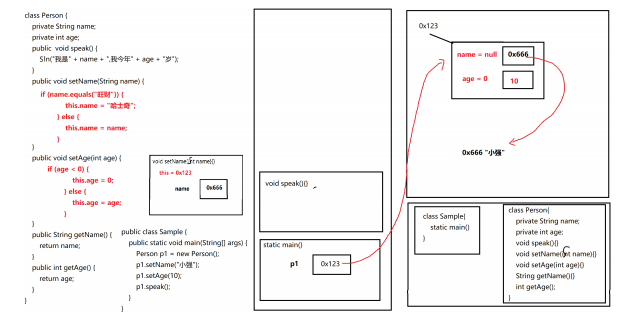1. javac 编译Sample.java源代码 生成Sample.class和Person.class两个字节码文件
2. 如果java Person ，运行Person字节码文件，则报错，没有主函数不是主类
3. 只能java Sample 运行Sample程序
4. 将相关的字节码（Sample.class Person.class）文件加载进JVM中内存下的方法区
5. 在方法区中Sample字节码所在的区域里，找主函数，将主函数的栈帧加载进栈内存开始运行
6. 开始执行主函数的第一句代码，创建Person对象
7. 堆内存中开辟一个空间并分配地址，在该空间中创建成员变量并默认初始化
8. 在主函数空间中创建局部变量p1，并将该对象的地址传给p1
9. 接着执行主函数第二句代码，调用p1对象的setName方法
10. 从方法区中的Person里，将setName函数栈帧加载进栈，主函数暂停运行
11. setName进栈后，创建局部变量name（形参），并将实参“小强”这个字符串在字符串常量池中的地址赋予name
12. 因为setName成员函数只有一份在方法区中Person所属区间里，之后可以被多个同类对象调用，为了区分到底是哪个对象调用的该方法，所以在每一个成员函数中，都会有一个隐藏的关键字数据 this ,this相当于一个变量来存储当前对象的地址。（当前对象的引用）
13. 执行setName中的内容，如果数据没有问题的话，就将局部变量的值赋值个当前对象的成员
变量
14. setName函数执行最后一行隐藏的return，表示函数结束并弹栈
15. 主函数成为当前栈顶，继续执行
16. 执行p1调用setAge函数，从方法区中Person所属空间里找setAge这一段代码，将该函数栈帧加载进栈内存成为新的栈顶，则主函数暂停，该函数运行。先创建形参age的局部变量，接收实
参传来的值10，为了区分对象的调用关系，自带this关键字数据，this存的还是p1的地址，如果age没有问题，则将10传给this所指向的对象中age这个成员变量。setAge执行最后一行隐藏的return，表示函数结束并弹栈
17. 主函数称为新的栈顶继续执行，调用p1的speak函数进栈
18. 在方法区中Person字节码所属空间里读取speak代码，将该栈帧加载进栈内存中，主函数暂停，该函数执行，无形参只能表示没有形参的局部变量，但是在函数内部也可以创建其他的局部变量，并且有this关键数据存的是p1的地址，然后去打印name和age，由于speak空间中已经没有其他名为name或age的局部变量，所以找不到，接着找this对象中的数据，找到了则打印。直至函数结束并弹栈
19. 主函数又称为栈顶，也没有代码了，执行隐藏的return，主函数弹栈，表示程序结束。

• 生命周期

• 存储位置

• 定义位置

• 初始化

## 7.5 构造函数

权限修饰符 类名(参数列表) {
构造函数的代码块
}

• 构造函数没有返回值
• 构造函数的名称必须是类名
• 参数列表可选的，构造函数是可以重载的
• 虽然构造函数没有返回值，还是存在return关键字的
• 当我们的类中没有定义任何构造函数时，会有一个默认隐藏的无参构造函数存在
• 构造函数和成员函数一样，为了区分对象的调用，构造函数自带this关键字数据

• 如果一旦定义其他参数列表的构造函数的话，这个隐藏的无参构造函数就会消失，所建议手写出来
• 构造函数只有在创建对象的时候执行，当对象创建完毕之后，该对象的构造函数则不能执行
• 成员函数只有在对象创建之后才能执行
• 成员函数能否直接调用构造函数？不能够的，报找不到符号错误 会误认为是同名的成员函数
• 构造函数能否直接调用成员函数呢？能够，但是 这些成员函数一般是构造函数的部分代码片段被切割出来了而已，从语意上而言，不属于对象的特有行为（也有特例），所以这些函数长得样子就是
成员函数的样子，但没有必要向外界提供访问，所以加上private
• 构造函数能否直接调用构造函数呢？可以，但是必须通过 this(参数列表) ，需要注意的是，构造函数可以单向调用其他构造函数，但坚决不能出现回调。
• 构造函数是在创建对象的时候执行的，可以在期间对成员变量进行初始化，问：setXXX还需要不？看需求，如果后期成员变量需要修改，则提供setXXX修改器
public class Sample {
public static void main(String[] args) {
Person p = new Person();//new 构造函数;
p.setName("旺财");
p.setAge(10);
p.speak();
Person p2 = new Person();//new 构造函数;
p2.setName("小强");
p2.setAge(20);
p2.speak();
Person p3 = new Person("如花",40);
p3.speak();
p3.test();
//p3.part();
Car car1 = new Car();
Car car2 = new Car(4);
Car car3 = new Car(4,"红色");
Car car4 = new Car(8,"武士黑",20);
car1.run();
car2.run();
car3.run();
car4.run();
}
}
class Car {
private int wheel = 4;
private String color;
private int weight;
public Car(){
}
public Car(int wheel) {
this(wheel,null,0);
}
public Car(int wheel,String color) {
this(wheel,color,0);
}
public Car(int wheel,String color,int weight) {
this.wheel = wheel;
this.color = color;
this.weight = weight;
//this();//递归构造器调用
}
public void run() {
System.out.println(wheel + ":" + color + ":" + weight);
}
//这个不是重载 Car(int)已经存在了(wheel)
/*
public Car(int weight) {
}
*/
}
class Person {
private String name;
private int age;
//这就是隐藏的构造函数
public Person() {
System.out.println("一个Person创建出来了！");
part();
part();
part();
part();
}
//语意
private void part() {
System.out.println("100行代码");
}
public Person(String name,int age) {
System.out.println("一个Person创建出来了！");
this.name = name;
this.age = age;
}
public void test() {
Person();//实际上这一段代码并不表示调用无参构造函数
//而表示去调用名字为Person的成员函数！
}
public void Person() {
System.out.println("没想到吧！");
}
public void speak() {
System.out.println(name + ":" + age);
}
public void setName(String name) {
this.name = name;
}
public void setAge(int age) {
this.age = age;
}
}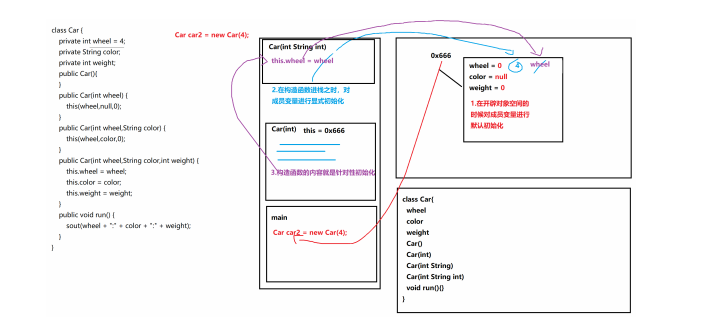## 7.6 对象的创建流程及内存图解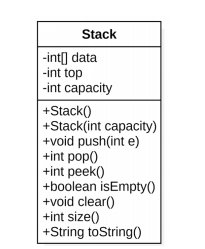public class Sample{
public static void main(String[] args) {
Stack stack = new Stack();
stack.pop();
System.out.println(stack);
for(int i=1; i<=10;i++){
stack.push(i);
}
System.out.println(stack);
stack.pop();
System.out.println(stack);
System.out.println(stack.peek());
stack.clear();
System.out.println(stack);
System.out.println(stack.peek());
}
}
class Stack{
private int[] data;
private int top = -1;
private static int capacity = 10;

public Stack() {
this(capacity);
}

public Stack(int capacity) {
data = new int[capacity];
}

public void push(int e){
if(size() == data.length) {
reSize(data.length);
}
data[++top] = e;
}

public void reSize(int length){
int[] arr = new int[length * 2];
for(int i=0; i<= top; i++) {
arr[i] = data[i];
}
data = arr;
}
public int pop(){
if(isEmpty()){
System.out.println(">>>栈为空，弹不出元素了！");
return -1;
}
int e = data[top];
top--;
if(data.length > capacity && size() == data.length / 4) {
reSize(data.length / 2);
}
return e;
}

public void clear(){
top = -1;
data = new int;
}
public int peek(){
if(isEmpty()){
System.out.println(">>>栈为空，无栈顶元素！");
return -1;
}
return data[top];
}

public boolean isEmpty(){
}

public int size() {
}

public String toString() {
if(isEmpty()){
return "[]";
}
String s = "[";
for(int i=0; i <= top; i++) {
if(i == top){
s += data[i] + "]";
}else{
s += data[i] + ", ";
}
}
return s;
}

}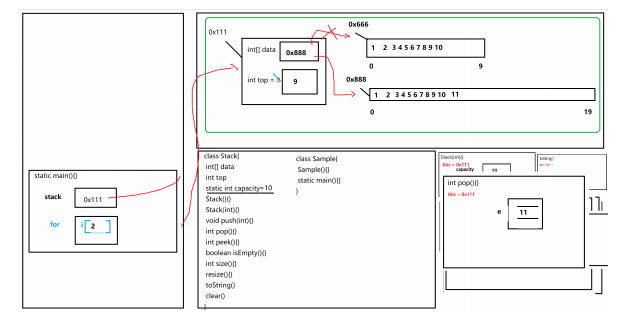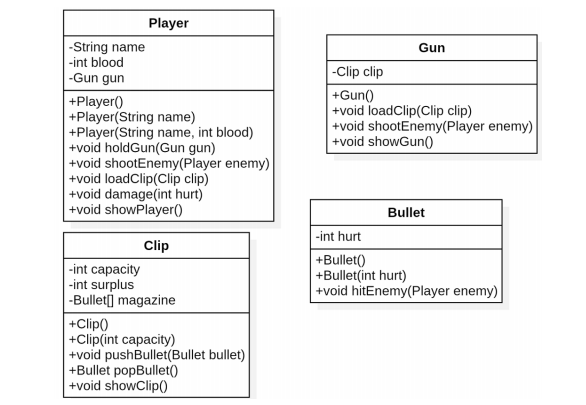public class Sample02{
public static void main(String[] args) {
Player p1 = new Player("老王",100);
Player p2 = new Player("老李",100);
p1.shootEnemy(p2);
Gun gun = new Gun();
p1.holdGun(gun);
p1.shootEnemy(p2);
Clip clip = new Clip();
for (int i = 1; i <= 30; i++) {
clip.pushBullet(new Bullet());
}
for (int i = 1; i <= 30; i++) {
p1.shootEnemy(p2);
}
p1.shootEnemy(p2);
}
}
//人
class Player{
//人物姓名
private String name;
//人物血值
private int blood;
//人物的枪
private Gun gun;

public Player() {}

//人物初始化
public Player(String name,int blood){
this(name,blood,null);
}

//人物初始化
public Player(String name,int blood,Gun gun){
this.name = name;
this.blood = blood;
this.gun = gun;
}

//设置枪
public void holdGun(Gun gun) {
this.gun = gun;
}

//攻击敌人
public void shootEnemy(Player enemy) {
if(gun == null) {
System.out.println(">>>玩家信息：没有枪，开P");
}else{
System.out.printf(">>>玩家信息：%s向%s开了一枪\n",name,enemy.name);
gun.shootEnemy(enemy);
}
}

//上弹夹
if(gun == null){
System.out.println(">>>玩家信息：没抢，装不了弹夹");
}else{
}
}

//玩家受到攻击
public void damage(int hurt) {
//血量为0，攻击不了
if(blood == 0) {
System.out.println(">>>玩家信息：" + name + "已经成盒，请勿鞭尸");
}else{
//否则扣血
blood -= hurt;
if(blood > 0){
System.out.println(">>>玩家信息：" + name + "掉血" + hurt + ",剩余" +blood);
}else{
//扣血小于0后，重新赋血值，输出信息
blood = 0;
System.out.println(">>>玩家信息：" + name + "已经成盒");
}
}

}

}
//枪
class Gun{
private Clip clip;

public Gun() {
this(null);
}
//初始化弹夹
public Gun(Clip clip) {
this.clip = clip;
}

//上弹夹
this.clip = clip;
}

//攻击敌人
public void shootEnemy(Player enemy) {
//判断枪是否有弹夹
if(clip == null) {
System.out.println(">>>枪信息：没有弹夹，开了个空枪");
return;
}
//弹夹弹出一颗子弹
Bullet bullet = clip.popBullet();
//弹出子弹为空，则弹夹没有子弹
if(bullet == null) {
System.out.println(">>>枪信息：弹夹没子弹 开了个空枪");
return;
}else{
bullet.hitEnemy(enemy);
}

}

}

//弹夹
class Clip{
//弹夹的容量
private int capacity = 30;
//弹夹剩余的子弹
private int surplus = 0;
//弹夹的子弹容器
private Bullet[] magazine;

public Clip(){
this(30);
}
//初始化子弹容器和弹夹的容量
public Clip(int capacity) {
this.capacity = capacity;
magazine = new Bullet[capacity];
}

//装入一颗子弹
public void pushBullet(Bullet bullet) {
//判断弹夹中子弹是否已经装满
if(surplus == capacity) {
System.out.println(">>>弹夹信息：弹夹已满，无法装入子弹");
return;
}else{
magazine[surplus++] = bullet;
showClip();
}
}

//弹出一颗子弹
public Bullet popBullet() {
//判断弹夹中是否有子弹
if(surplus == 0){
System.out.println(">>>弹夹信息：弹夹为空，无法弹出子弹！");
return null;
}else {
//从子弹容器中取出一颗子弹
Bullet bullet = magazine[surplus-1];
//剩余子弹--
surplus--;
showClip();
return bullet;
}
}

public void showClip() {
System.out.printf(">>>弹夹信息：%d/%d\n",surplus,capacity);
}
}
//子弹
class Bullet{
//子弹的伤害
private int hurt;

public Bullet() {}

//为子弹赋特殊伤害
public Bullet(int hurt) {
this.hurt = hurt;
}

//子弹伤害敌人
public void hitEnemy(Player enemy) {
enemy.damage(hurt);
}

}


## 7.7 static关键字

• 静态函数一旦定义出来，可以直接用类名去调用,当然也可以通过创建对象来去调用静态函数！
• 静态优先于对象存在，且在同一类中，静态无法访问非静态（成员），非静态是可以访问静态
• 静态函数中不存在this()

1.当通过对象去调用一个属性时，先找成员，再找静态，最后找父类
2.如果从成员函数中去调用一个属性时，先找局部，再找成员，再找静态，最后找父类

• 节省堆内存中的空间
• 可以不用费力气去创建对象来调用功能
• 可以对类进行一些初始操作（结合代码块来做）
import java.util.Scanner;
public class Demo {
public static void main(String[] args) {
Chinese c1 = new Chinese();
Chinese c2 = new Chinese();
Chinese c3 = new Chinese();
System.out.println(c1.country);
System.out.println(Chinese.country);
c1.show();
Chinese.show();
/*
Chinese.test();
*/
}
}
class Chinese {
String name;
int age;
static String country;
//静态代码块
static {
country = "China";
System.out.println("init....");
}
Chinese() {
System.out.println("Chinese....");
}
public void test(){
int num = 10;
System.out.println(num + name + country);
}
public static void show() {
/*
//无法从静态上下文中引用非静态 方法 test()
test();
//无法从静态上下文中引用非静态 变量 name
System.out.println("show...." + name);
*/
}
}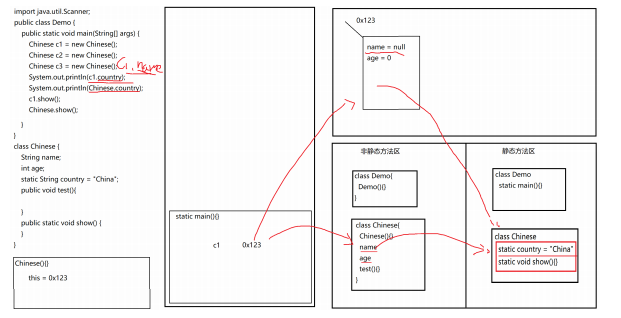## 7.8 静态变量与成员变量

• 成员变量存储在堆内存中对象所属空间里
• 静态变量存储在静态方法区中对应的字节码空间里

• 成员变量随着对象的创建而创建，随着对象的消亡而消亡
• 静态变量随着类的加载而存在，随着程序的结束而消失

• 成员变量属于对象的，称之为是对象的特有属性
• 静态变量属于类的，称之为是类的属性，或者叫对象的共有属性

• 成员变量在外界必须通过创建对象来调用，内部的话成员函数可以直接调用成员变量，但是静态函数不能直接调用成员变量，如果非要在静态函数中调用成员的话，只能创建对象，通过对象来调用

• 静态变量在外界可以通过对象调用，也可以通过类来调用，内部的话静态函数/成员函数可以调用静态变量

public class Demo {
public static void main(String[] args) {
//StackOverFlowError 栈内存溢出
A a = new A();
System.out.println(a == a.a);
System.out.println(a.a == a.a.a);
}
}
class A {
//OutOfMemoryError 堆内存溢出
int[][] arr = new int;
A a = new A();
}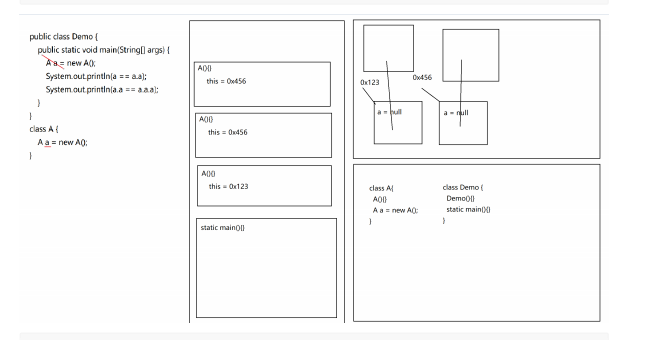public class Demo {
public static void main(String[] args) {
A a = new A();
System.out.println(a == a.a);
System.out.println(a.a == a.a.a);
}
}
class A {
static A a = new A();
}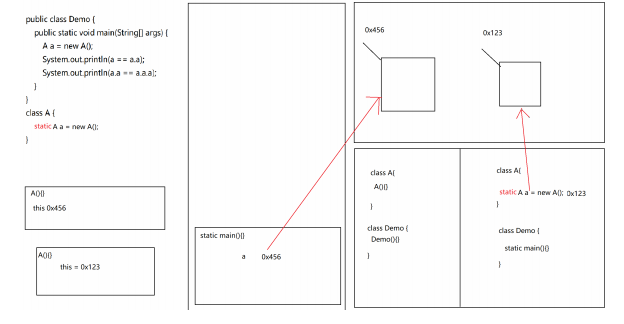## 7.9 java对象初始化顺序

1. 父类静态代码块，父类静态成员变量（同级，按代码顺序执行）
2. 子类静态代码块，子类静态成员变量（同级，按代码顺序执行）
3. 父类普通代码块，父类普通成员变量（同级，按代码顺序执行）
4. 父类构造方法
5. 子类普通代码块，子类普通成员变量（同级，按代码顺序执行）
6. 子类构造方法

注意点

1. 静态内容只在类加载时执行一次，之后不再执行。
2. 默认调用父类的无参构造方法，可以在子类构造方法中利用super指定调用父类的哪个构造方法。

class Father {
public Father() {
System.out.println("父类无参构造方法");
}
static {
System.out.println("父类静态代码块1");
}
private static int a = Help.fatherStaticMemberVarInit();
static {
System.out.println("父类静态代码块2");
}
{
System.out.println("父类普通代码块1");
}
private int b = Help.fatherMemberVarInit();
{
System.out.println("父类普通代码块2");
}
public Father(int v) {
System.out.println("父类带参构造方法");
}
}

class Son extends Father {
static {
System.out.println("子类静态代码块1");
}
private static int a = Help.sonStaticMemberVarInit();
static {
System.out.println("子类静态代码块2");
}
{
System.out.println("子类普通代码块1");
}
private int b = Help.sonMemberVarInit();
{
System.out.println("子类普通代码块2");
}
public Son() {
// super(2018);
System.out.println("子类构造方法");
}
}

class Help {
public static int fatherStaticMemberVarInit() {
System.out.println("父类静态成员变量");
return 0;
}
public static int fatherMemberVarInit() {
System.out.println("父类普通成员变量");
return 0;
}
public static int sonStaticMemberVarInit() {
System.out.println("子类静态成员变量");
return 0;
}
public static int sonMemberVarInit() {
System.out.println("子类普通成员变量");
return 0;
}
}

public class Test1 {
public static void main(String[] args) {
Son son1 = new Son();
System.out.println("===================");
Son son2 = new Son();
}
}
/*

===================

*/


## 编程练习题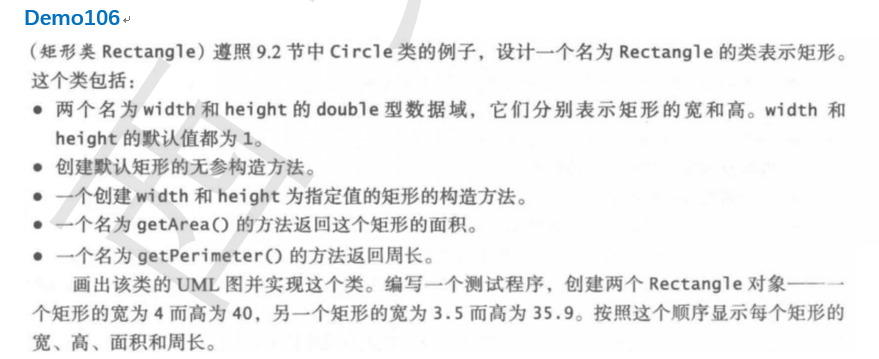public class Demo106{
public static void main(String[] args){
Rectangle r1 = new Rectangle();
System.out.println(r1.getArea());
System.out.println(r1.getPermeter());
Rectangle r2 = new Rectangle(4.0,5.0);
System.out.println(r2.getArea());
System.out.println(r2.getPermeter());
}
}
class Rectangle{
private double width = 1;
private double height = 1;

public Rectangle(){}

public Rectangle(double width,double height){
this.width = width;
this.height = height;
}

public double getArea(){
return width * height;
}

public double getPermeter(){
return 2 * (width + height);
}
}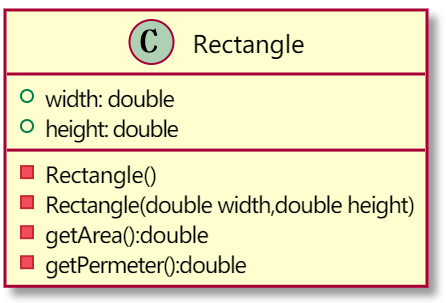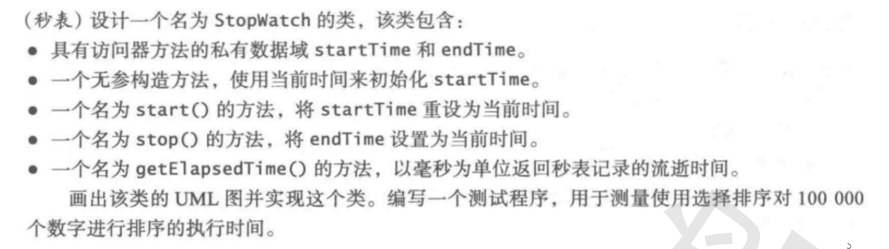public class Demo107 {
public static void main(String[] args) {

StopWatch sw = new StopWatch();
sw.start();
for(int i = 0; i<1000000; i++){

}
sw.stop();
System.out.println(sw.getElapsedTime());

}
}
class StopWatch {
private long startTime;
private long endTime;
public StopWatch(){
startTime = System.currentTimeMillis();
}

public void start() {
startTime = System.currentTimeMillis();
}

public void stop() {
endTime = System.currentTimeMillis();
}

public long getElapsedTime() {
return endTime - startTime;
}
}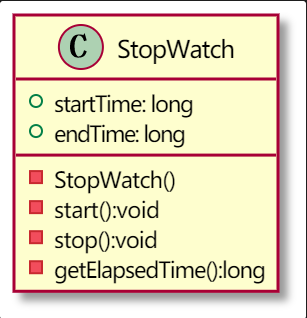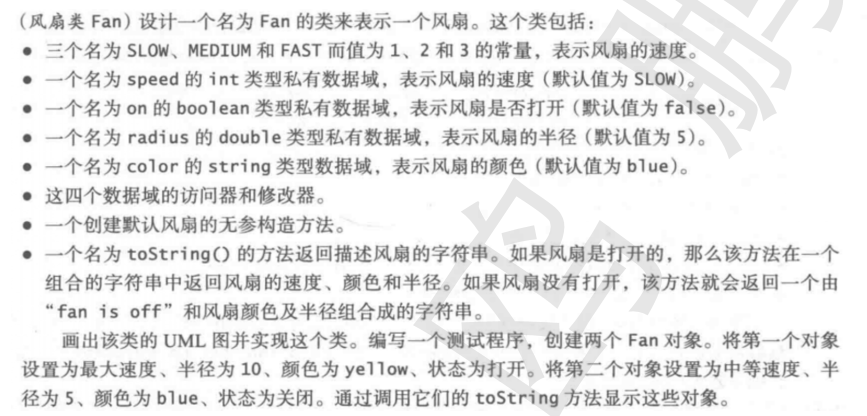public class Demo108 {
public static void main(String[] args) {
Fan f1 = new Fan();
System.out.println(f1);

f1.setOn(true);
f1.setSpeed(Fan.FAST);
f1.setColor("red");
System.out.println(f1);
}
}
class Fan {
public static final int SLOW = 1;
public static final int MEDIUM = 2;
public static final int FAST = 3;

private int speed = SLOW;
private boolean on = false;
private String color = "blue";

public Fan(){}

public void setSpeed(int speed){
this.speed = speed;
}

public int getSpeed(){
return this.speed;
}

public void setOn(boolean on){
this.on = on;
}

public boolean isOn(){
return this.on;
}

}

}

public void setColor(String color){
this.color = color;
}

public String getColor(){
return this.color;
}

public String toString(){
if(on){
return "The Fan speed is "+getSpeed()+" color is "+getColor()+" radius is "+getRadius();
}else{
return "Fan is off and the color is "+getColor()+" radius is "+getRadius();
}

}
}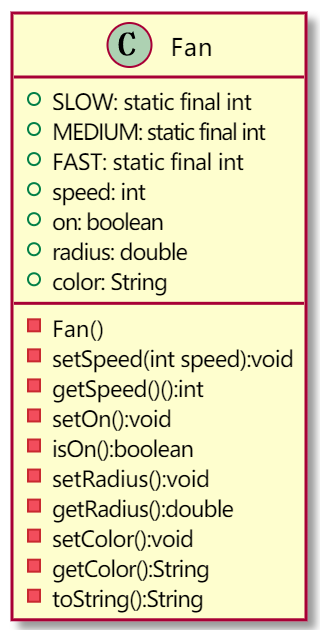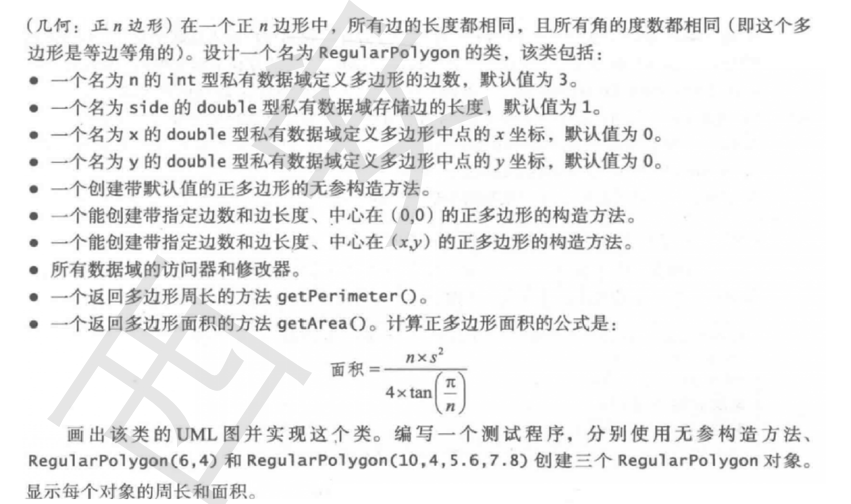public class Demo109 {
public static void main(String[] args) {
RegularPolygon r1 = new RegularPolygon();
System.out.println(r1.getArea());
System.out.println(r1.getPerimeter());

RegularPolygon r2 = new RegularPolygon(5,6,5,6);
System.out.println(r2.getArea());
System.out.println(r2.getPerimeter());
}
}
class RegularPolygon {
private int n = 3;
private double side = 1;
private double x = 0;
private double y = 0;

public RegularPolygon(){}

public RegularPolygon(int n,double side) {
this.n = n;
this.side = side;
}

public RegularPolygon(int n,double side,double x,double y) {
this.n = n;
this.side = side;
this.x = x;
this.y = y;
}

public double getPerimeter(){
return n * side;
}

public double getArea() {
return n * Math.pow(side,2) / (4 * Math.tan(Math.PI / n));
}

public void setN(int n){
this.n = n;
}

public int getN(){
return this.n;
}

public void setSide(double side){
this.side = side;
}

public double getSide(){
return this.side;
}

public void setX(double x){
this.x = x;
}

public double getX(){
return this.x;
}

public void setY(double y){
this.y = y;
}

public double getY(){
return this.y;
}
}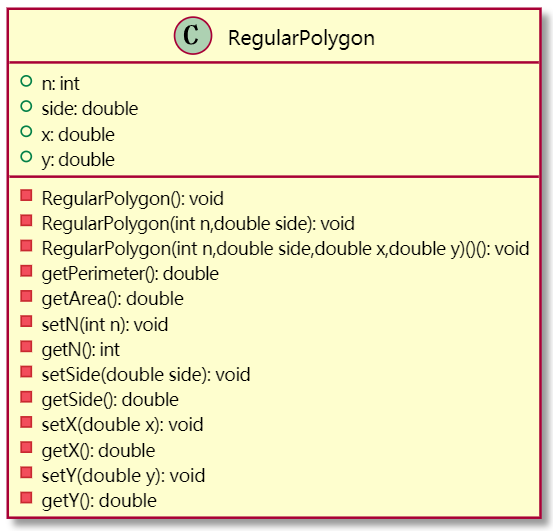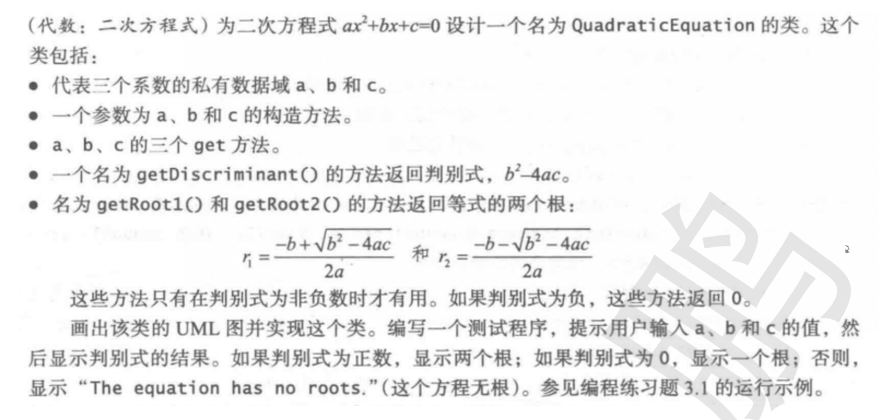public class Demo110{
public static void main(String[] args) {
System.out.println(q.getRoot1());
System.out.println(q.getRoot2());
}
}
private double a;
private double b;
private double c;

this.a = a;
this.b = b;
this.c = c;
}

public double getDiscriminant(){
double result = Math.pow(b,2) - 4 * a * c;
if(result < 0){
return 0;
}
return result;
}

public double getRoot1() {
return (-b + Math.sqrt(getDiscriminant())) / 2 * a;
}

public double getRoot2() {
return (-b - Math.sqrt(getDiscriminant())) / 2 * a;
}

public double getA(){
return this.a;
}

public void setA(double a){
this.a = a;
}

public double getB(){
return this.b;
}

public void setB(double b){
this.b = b;
}

public double getC(){
return this.c;
}

public void setC(double c){
this.c = c;
}
}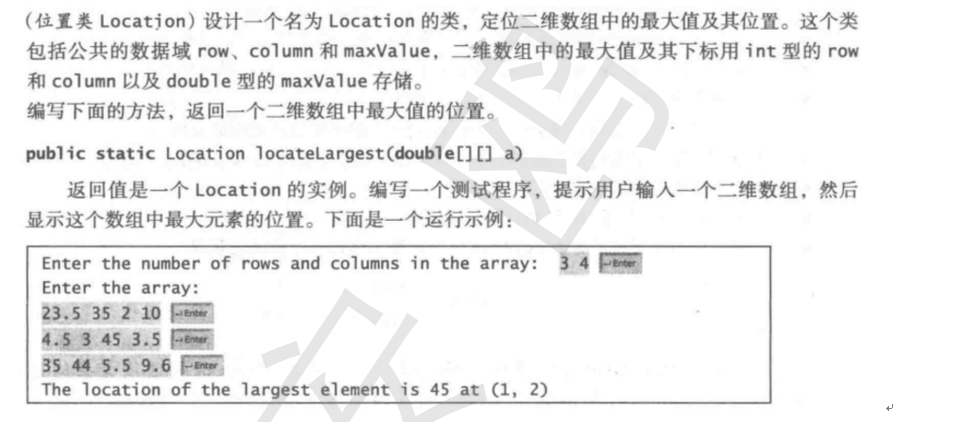import java.util.*;
public class Demo111{
public static void main(String[] args){
System.out.print("Enter the number of rows and columns in the array: ");
Scanner input = new Scanner(System.in);
int row = input.nextInt();
int column = input.nextInt();

double [][] array = new double[row][column];
System.out.println("Enter the array:");
for(int i =0; i<array.length;i++){
for(int j=0;j<array[i].length;j++){
array[i][j] = input.nextDouble();
}
}
Location l = new Location();
Location a = l.locateLargest(array);
System.out.println("The location of the largest element is "+a.getMax()+" at ("+a.getRow()+", "+a.getColume()+")");
}
}
class Location{
private int row;
private int column;
private double maxValue;

public int getRow(){
return this.row;
}

public void setRow(int row){
this.row = row;
}

public double getMax(){
return this.maxValue;
}

public void setMax(double maxValue){
this.maxValue = maxValue;
}

public int getColume(){
return this.column;
}

public void setColume(int column){
this.column = column;
}

public static Location locateLargest(double[][] a){
int row = 0,column = 0;
double maxValue = 0;
for(int i = 0; i<a.length; i++){
for(int j = 0;j<a[i].length;j++){
maxValue = a[i][j] > maxValue ? a[i][j] : maxValue;
row = i;
column = j;
}
}
Location l = new Location();
l.row = row;
l.column =column;
l.maxValue = maxValue;
return l;
}
}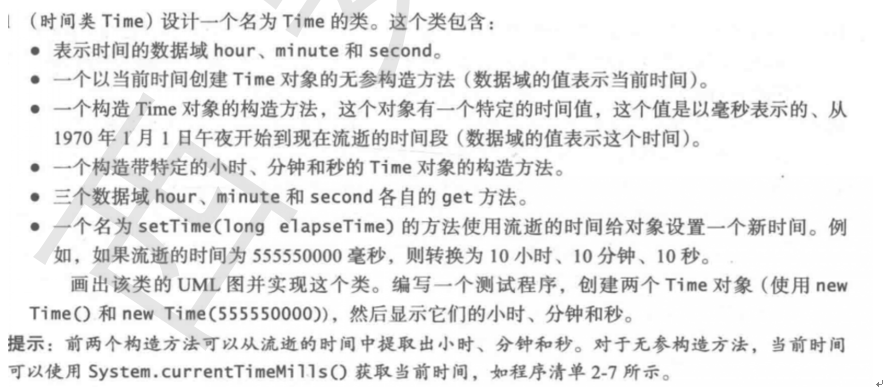public class Demo112 {
public static void main(String[] args) {
Time t1 = new Time();
System.out.println(t1.toString());
Time t2 = new Time(120233131L);
System.out.println(t2.toString());
Time t3 = new Time(12,30,56);
System.out.println(t3.toString());
t3.setTime(System.currentTimeMillis());
System.out.println(t3.toString());
}
}
class Time {
private long hour;
private long minute;
private long second;

public Time() {
this(System.currentTimeMillis());
}

public Time(long millis) {
hour = cacuHour(millis);
minute = cacuMinute(millis);
second = cacuSecond(millis);
}

public long getHour(){
return hour;
}
public long getMinute(){
return minute;
}
public long getSecond(){
return second;
}

public void setTime(long elapseTime) {
hour = cacuHour(elapseTime);
minute = cacuMinute(elapseTime);
second = cacuSecond(elapseTime);
}

public String toString(){
return hour+":"+minute+":"+second;
}

public Time(long hour,long minute,long second) {
this.hour = hour;
this.minute = minute;
this.second = second;
}

private long cacuHour(long millis) {
return millis / 1000 / 60 / 60 % 24 + 8;
}

private long cacuMinute(long millis) {
return millis / 1000 / 60 % 60;
}

private long cacuSecond(long millis) {
return millis / 1000 % 60;
}
}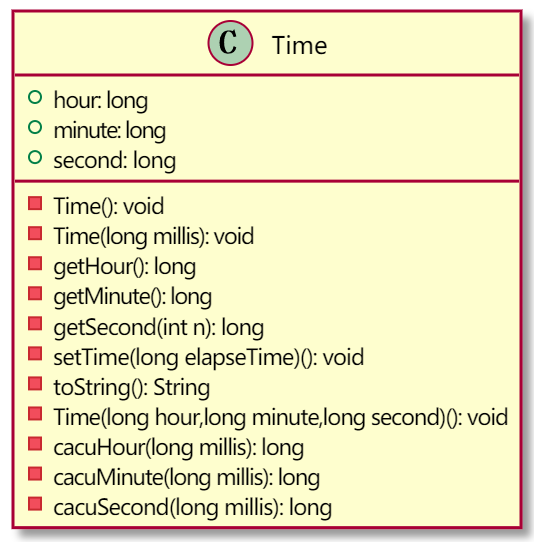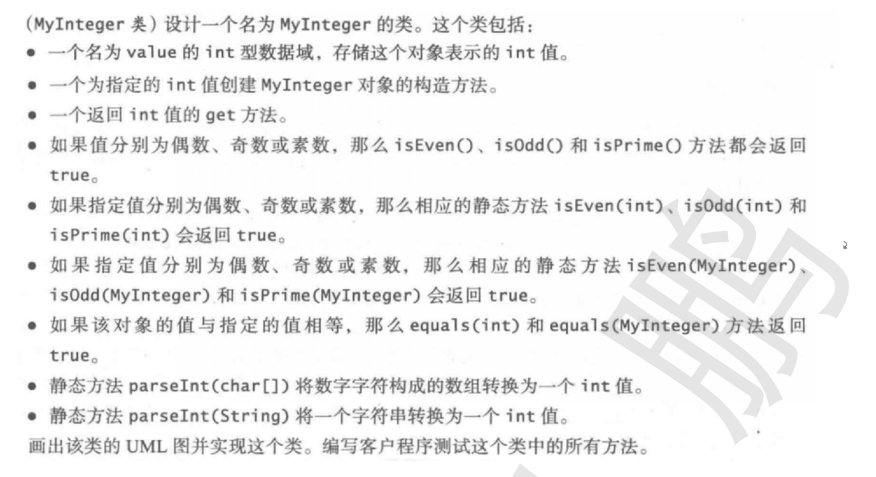public class Demo113 {
public static void main(String[] args) {
MyInteger m1 = new MyInteger(3);
System.out.println(m1.isEven());
System.out.println(m1.isOdd());
System.out.println(m1.isPrime());
MyInteger m2 = new MyInteger(4);
MyInteger m3 = new MyInteger(13);
System.out.println(MyInteger.isEven(m2));
System.out.println(MyInteger.isPrime(m3));
System.out.println(m2.equals(m3));
System.out.println(MyInteger.parseInt("1234") + 1);
}
}
class MyInteger {
private int value;
public MyInteger(int value) {
this.value = value;
}
public int get() {
return value;
}
public boolean isEven() {
return value % 2 == 0;
}
public boolean isOdd() {
return value % 2 == 1;
}
public boolean isPrime() {
for (int i = 2; i <= value / 2; i++) {
if (value % i == 0) {
return false;
}
}
return true;
}
public static boolean isEven(MyInteger integer) {
return integer.get() % 2 == 0;
}
public static boolean isOdd(MyInteger integer) {
return integer.get() % 2 == 1;
}
public static boolean isPrime(MyInteger integer) {
for (int i = 2; i <= integer.get() / 2; i++) {
if (integer.get() % i == 0) {
return false;
}
}
return true;
}
public boolean equals(int num) {
return value == num;
}
public boolean equals(MyInteger integer) {
return value == integer.get();
}
public static int parseInt(String str) {
int result = 0;
for (int i = 0; i < str.length(); i++) {
int num = str.charAt(i) - '0';
result = num + result * 10;
}
return result;
}
}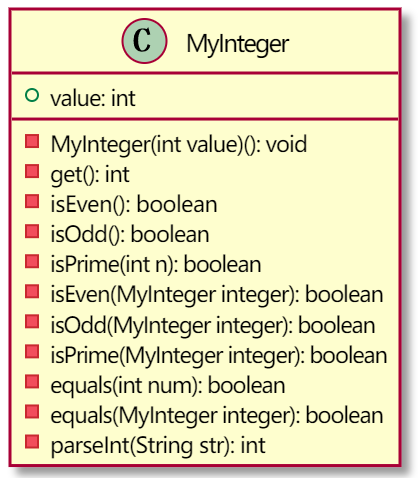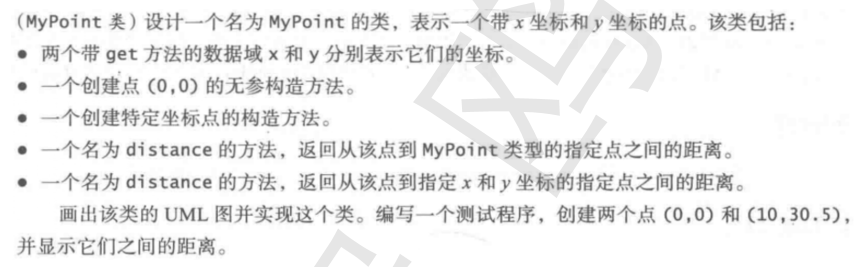public class Demo114 {
public static void main(String[] args) {
MyPoint p1 = new MyPoint();

MyPoint p2 = new MyPoint(10,30.5);

System.out.println(p1.distance(p2));
System.out.println(p2.distance(11,11));
}
}
class MyPoint {
private double x = 0;
private double y = 0;

public MyPoint(){}

public MyPoint(double x, double y){
this.x = x;
this.y = y;
}

public double distance(MyPoint point){
return Math.hypot(point.getX() - x,point.getY() - y);
}

public double distance(double x, double y){
return Math.hypot(x - this.x,y - this.y);
}

public double getX(){
return x;
}

public double getY(){
return y;
}
}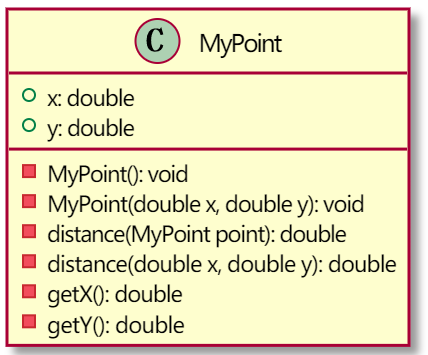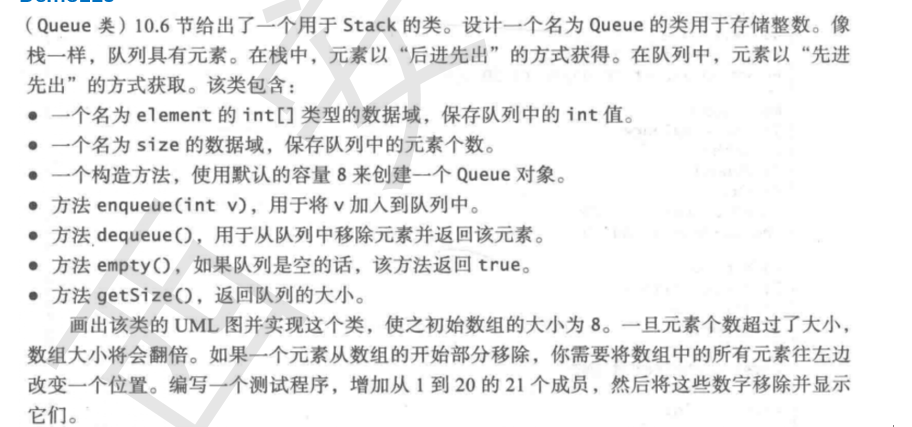public class Demo115 {
public static void main(String[] args) {
Queue q1 = new Queue();
System.out.println(q1);
//遍历添加10个元素，队列进行扩容
for(int i=1; i <= 10;i++){
q1.enqueue(i);
}
System.out.println(q1);
//队列出6个元素，此时有效元素的个数小于队列的1/4大小，进行缩容
for(int i=1;i<=6;i++){
System.out.println(q1.dequeue());
System.out.println(q1);
}
System.out.println("当前队列的有效元素个数为："+q1.getSize());
}
}
class Queue {
//队列容器用于存储元素，element.length == size 表示队列已满
private int[] element;
//队列中有效元素的个数
private int size;
//队列容器的默认值
private int capacity = 8;

//默认队列初始化为8
public Queue(){
element =new int[capacity];
size = 0;
}

//向队列添加一个元素
public void enqueue(int v) {
//先判断该队列是否已经满了，满了的话对其进行扩容1倍
if(size == element.length){
//扩容1倍
resize(element.length * 2);
}
//扩容还是没用扩容，都要将元素添加进队列，size要自增
element[size++] = v;
}

//删除并返回队列的第一个元素
public int dequeue() {
int result = element;
//当删除元素后队列的有效长度为当前队列的四分之一时，对队列进行缩容,并且默认缩容到最小为8
if(size <= element.length / 4 && element.length > capacity){
//对队列缩容二分之一，保证在入队时进行不必要的扩容，留有容量进行增加元素
resize(element.length / 2);
}
for(int i=1; i<size;i++){
element[i-1] = element[i];
}
//队列出队一个元素size要减1
size--;
return result;
}

//扩容和缩容函数
private void resize(int len) {
int[] newArray = new int[len];
//遍历原数组进行复制元素到新的数组
for(int i=0; i<size;i++) {
newArray[i] = element[i];
}
element = newArray;
}

//打印队列中的元素
public String toString(){
String str = "[";
//判断队列是否为空
if(isEmpty()){
return str += "]";
}else{
//遍历数组打印内容，并判断是否为最后一个元素，是的话就加]，否则就加，
for(int i=0; i<size; i++){
if(i == size - 1){
str += element[i]+"]";
}else{
str += element[i]+",";
}
}
}
return str;
}

//判断队列是否为空
public boolean isEmpty() {
return size == 0;
}

//返回队列的大小
public int getSize() {
return size;
}
}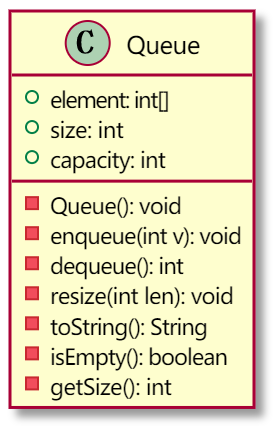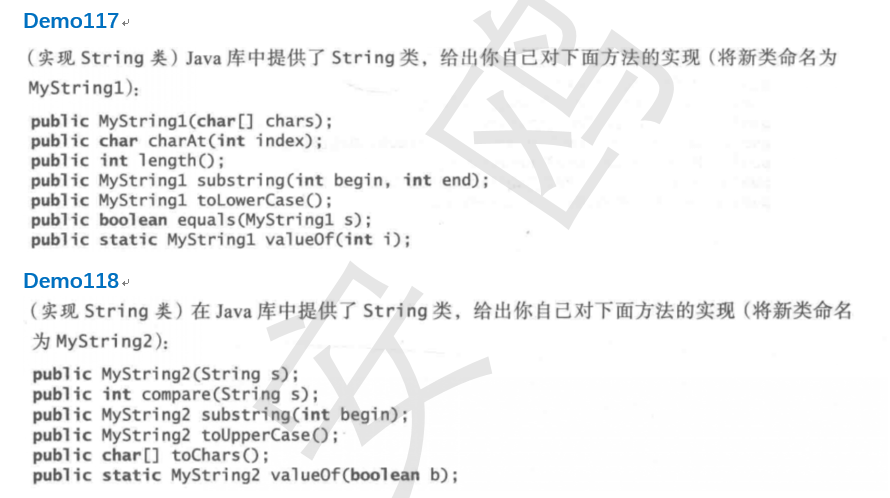public class Demo117 {
public static void main(String[] args) {
char[] ch = {'a','b','c'};
MyString s1 = new MyString(ch);
MyString s2 = new MyString("abg");
System.out.println(s1.compareTo(s2));
MyString s3 = new MyString("PPviuiNN");
s3.toLowerCase().show();
s1.toUpperCase().show();
System.out.println(s2.compareToIgnoreCase(s3));
s3.concat(s1).show();
System.out.println(s1.contains(s2));

MyString s4 = new MyString("xxx.mp4");
System.out.println(s4.endsWith(new MyString(".mp4")));

System.out.println(s4.equals(new MyString("xxx.mp")));
System.out.println(s4.equalsIgnoreCase(new MyString("xxx.MP4")));
System.out.println(s4.indexOf('m'));
System.out.println(s4.lastIndexOf('x'));
System.out.println(s3.indexOf(new MyString("iui")));
System.out.println(s3.lastIndexOf(new MyString("iui")));
s3.replace('P','a').show();
System.out.println(s3.startWith(new MyString("PP")));
s3.substring(2,5).show();
}
}
class MyString {
//定义一个字符数组来存放字符串
private char[] data;

public MyString(char[] chars) {
//将外部的字符数组，传递给内部的字符数组
data = new char[chars.length];
for(int i=0; i<chars.length; i++) {
data[i] = chars[i];
}
}

//将外部字符串传递给内部的data
public MyString(String s) {
data = new char[s.length()];
for(int i=0; i<s.length();i++){
data[i] = s.charAt(i);
}
}

//截取字符串
public MyString substring(int beginIndex,int endIndex){
char[] ch = new char[endIndex-beginIndex];
int index = 0;
for(int i=beginIndex;i<endIndex;i++){
ch[index++] = data[i];
}
return new MyString(ch);
}

//判断是否以字符开头
public boolean startWith(MyString s){
//定义两个指针，分别判断两个字符串的首字母是否相等，相等就继续判断
//判断到有一个字符串溢出为止，有溢出就返回true，否则就为false
int i = 0;
int j = 0;
while(true){
if(charAt(i) == s.charAt(j)){
i++;
j++;
if(j >= s.length()){
return true;
}
}else{
return false;
}
}
}

//替换字符串中的字符
public MyString replace(char oldChar,char newChar){
char[] chars = new char[length()];
for(int i=0;i<length();i++){
if(charAt(i) == oldChar){
chars[i] = newChar;
}else{
chars[i] = data[i];
}
}
return new MyString(chars);
}

//返回第一个字符相等的角标
public int indexOf(char c){
for(int i=0;i<length();i++){
if(charAt(i) == c){
return i;
}
}
return -1;
}

//返回最后一个字符相等的角标
public int lastIndexOf(char c){
for(int i=length()-1;i>=0;i--){
if(charAt(i) == c){
return i;
}
}
return -1;
}

//返回字符串的第一个角标
public int indexOf(MyString s){
//将两个数组变为局部变量，方便比较
char[] ch1 = data;
char[] ch2 = s.data;
//循环数组，每次比较两个字符串的首字母是否相等，相等的就遍历第二个字符串
//并与接下去的第一个字符串的字符比较，只要有一个不相等就返回-1,否则就返回i对应的角标
for(int i=0;i<length() - ch2.length;i++){
if(ch1[i] == ch2){
int j = i+1;
for(int l=1;l<ch2.length;l++,j++){
if(ch1[j] != ch2[l]){
return -1;
}
}
return i;
}
}
return -1;
}

//返回字符串相等的最后的一个角标
public int lastIndexOf(MyString s){
//将两个数组变为局部变量，方便比较
char[] ch1 = data;
char[] ch2 = s.data;
//循环数组，每次比较两个字符串的首字母是否相等，相等的就遍历第二个字符串
//并与接下去的第一个字符串的字符比较，只要有一个不相等就返回-1,否则就返回i对应的角标
for(int i = ch1.length-1;i>=ch2.length-1;i--){
if(ch1[i] == ch2[ch2.length-1]){
int j = i -1;
for(int l=ch2.length-2;l>=0;l--,j--){
if(ch1[j] != ch2[l]){
return -1;
}
}
return j+1;
}
}
return -1;
}

//判断两个字符串是否相等忽略大小写
public boolean equalsIgnoreCase(MyString s){
return compareToIgnoreCase(s) == 0;
}

//判断两个字符串是否相等
public boolean equals(MyString s){
return compareTo(s) == 0;
}

//判断字符串是否以xx结尾
public boolean endsWith(MyString s) {
//都从字符串的最后一个字符开始遍历
//判断两个字符是否相等，不相等返回false,直到有一个字符遍历完了后就返回true
int i = length() - 1;
int l = s.length() - 1;
while(true){
if(charAt(i) == s.charAt(l)){
i--;
l--;
if(l<0){
return true;
}
}else{
return false;
}
}
}

//判断一个字符串是否包含另一个字符串
public boolean contains(MyString s){
//将两个数组变为局部变量，方便比较
char[] ch1 = data;
char[] ch2 = s.data;
//循环数组，每次比较两个字符串的首字母是否相等，相等的就遍历第二个字符串
//并与接下去的第一个字符串的字符比较，只要有一个不相等就返回false,否则就返回true
for(int i=0;i<length() - ch2.length;i++){
if(ch1[i] == ch2){
int j = i+1;
for(int l=1;l<ch2.length;l++,j++){
if(ch1[j] != ch2[l]){
return false;
}
}
return true;
}
}
return false;
}

//连接两个字符串
public MyString concat(MyString s) {
//创建一个行的数组，长度为两个字符串的总和
//分别遍历两个数组，将其内容复制到新的数组中返回即可
char[] chars = new char[length() + s.length()];
int index =0;
for(int i=0;i<length();i++){
chars[index++] = data[i];
}
for(int i=0; i<s.length();i++){
chars[index++] = s.charAt(i);
}
return new MyString(chars);
}

//比较两个字符串的大小，忽略大小写
public int compareToIgnoreCase(MyString s) {
//将两个字符串都转为小写的字符串，在比较大小
MyString s1 = toLowerCase();
MyString s2 = s.toLowerCase();
return s1.compareTo(s2);

}

//将字符串中大写的字母转换为小写的字母
public MyString toLowerCase() {
//创建一个新的字符数组来存放转变后的字符
char[] chars = new char[length()];
for(int i=0; i<length();i++){
char ch = data[i];
if(isUpperLetter(ch)){
//将大写的字母转换为小写的字母
chars[i] = (char) (ch + 32);
}else{
//不是字母就直接赋值
chars[i] = ch;
}
}
return new MyString(chars);
}

//转换为大写字母
public MyString toUpperCase() {
char[] chars = new char[length()];
for(int i=0; i<length();i++){
char ch = data[i];
if(isLowerLetter(ch)){
chars[i] = (char) (ch - 32);
}else{
chars[i] = ch;
}
}
return new MyString(chars);
}

//判断是否为小写的字母
public boolean isLowerLetter(char ch){
return ch >= 'a' && ch <= 'z';
}

//判断是否为大写的字母
public boolean isUpperLetter(char ch){
return ch >= 'A' && ch <= 'Z';
}

//比较两个字符串的大小
public int compareTo(MyString s){
int i = 0;
int j = 0;
//定义左右指针，如果比较两个字符，如果相等就增加，有一个不等就返回两字符的差值，
//如果有一个字符已经遍历过了最大的角标就返回两个字符串长度的差值
while(true) {
if(charAt(i) == s.charAt(j)){
i++;
j++;
if(i == length() && j == s.length()){
return 0;
}
if(i < length() && j>=s.length() || i >= length() && j < s.length()){
return length() - s.length();
}
}else{
return charAt(i) - s.charAt(j);
}
}
}

//打印字符串
public void show() {
for(int i=0;i<length();i++){
System.out.print(data[i]);
}
System.out.println();
}

//根据字符索引返回字符
public char charAt(int index) {
return data[index];
}

//将MyString的类型的字符串赋值到data中
public MyString(MyString str) {
data = new char[str.length()];
for(int i=0; i<str.length();i++){
data [i] = str.charAt(i);
}
}

//返回字符数组的长度
public int length() {
return data.length;
}

}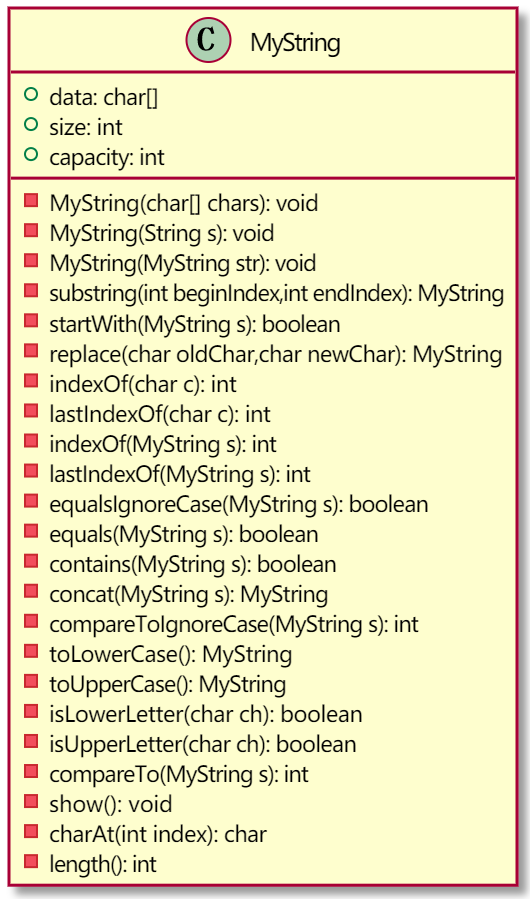# 八、继承

## 8.1 继承概述

### 8.1.1 继承概述

class Student extends Person{
void study() {
System.out.println("study....");
}
}
class Worker extends Person{
void work() {
System.out.println("work....");
}
}
class Teacher extends Person {
void teach() {
System.out.println("teach....");
}
}
class Person {
String name;
int age;
}


• 提高代码的复用性
• 类与类之间产生了关系，是多态的前提

Java中 类与类之间只能支持单继承接口与接口之间可以支持多继承

• 继承和传统的理解稍微有一些不同的，子类并不是或是父类的一个子集！实际上，一个子类通常比它的父类包含更多的信息和方法。（子类更多的是父类的一种升级和延伸）
• 父类中的一些私有内容，子类是获取不到的。如果父类对其自身的私有内容设置了公共的访问器和修改器的话，子类可以通过该访问器和修改器来获取父类的私有内容。

### 8.1.2 子父类中，成员变量的特点

1. 子类没有，父类有且非私有，子类对象能获取父类的属性
2. 子类没有，父类有且私有，子类对象不能获取父类的属性
3. 子类有，父类有且非私有，子类对象获取的是子类的属性，不存在重写的概念
4. 子类有，父类没有， 子类对象获取的是子类的属性
5. 如果子类中，成员变量父类变量局部变量重名时，在子类的方法中首先获取的是子类中的局部变量，使用this来获取子类的成员变量，使用super来获取父类空间中的父类变量。
public class Sample {
public static void main(String[] args) {
Zi zi = new Zi();
System.out.println(zi.num);
zi.show();
}
}
class Fu {
int num = 10;
}
//父类 超类 基类
class Zi extends Fu {
int num = 20;
void show() {
int num = 30;
System.out.println(num + "," + this.num + "," + super.num);
}
}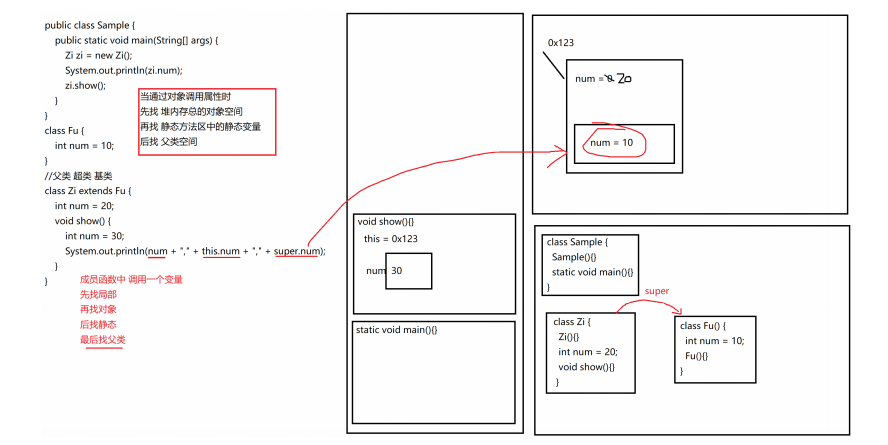### 8.1.3 子父类中，构造函数的特点

1. 构造方法的名字是与类名一致的。所以子类是无法继承父类构造方法的。
2. 构造方法的作用是初始化成员变量的。所以子类的初始化过程中，必须先执行父类的初始化动作。子类的构造方法中默认有一个 super() ，表示调用父类的构造方法，父类成员变量初始化后，才可以给子类使用。

• 每一个类中的构造函数第一句如果不是 this()调用本类中其他构造函数的话，默认第一句是隐藏的 super()
• 当创建子类对象时，在子类的构造函数执行之前，父类的构造函数先执行，是因为在创建子类对象的时候，需要父类为继承给子类的一些数据进行初始化
public class Sample {
public static void main(String[] args) {
Zi zi = new Zi();
}
}
class Fu {
int num;
Fu() {
System.out.println("Fu show..." + num);
num = 4;
}
}
class Zi extends Fu {
Zi() {
super(); //调用父类的无参构造函数 默认是隐藏的
//父类的构造函数一旦执行完毕 则紧接着执行子类成员变量的显示初始化
System.out.println("Zi show..." + num);
}
}
/*

Fu show...0
Zi show...4
*/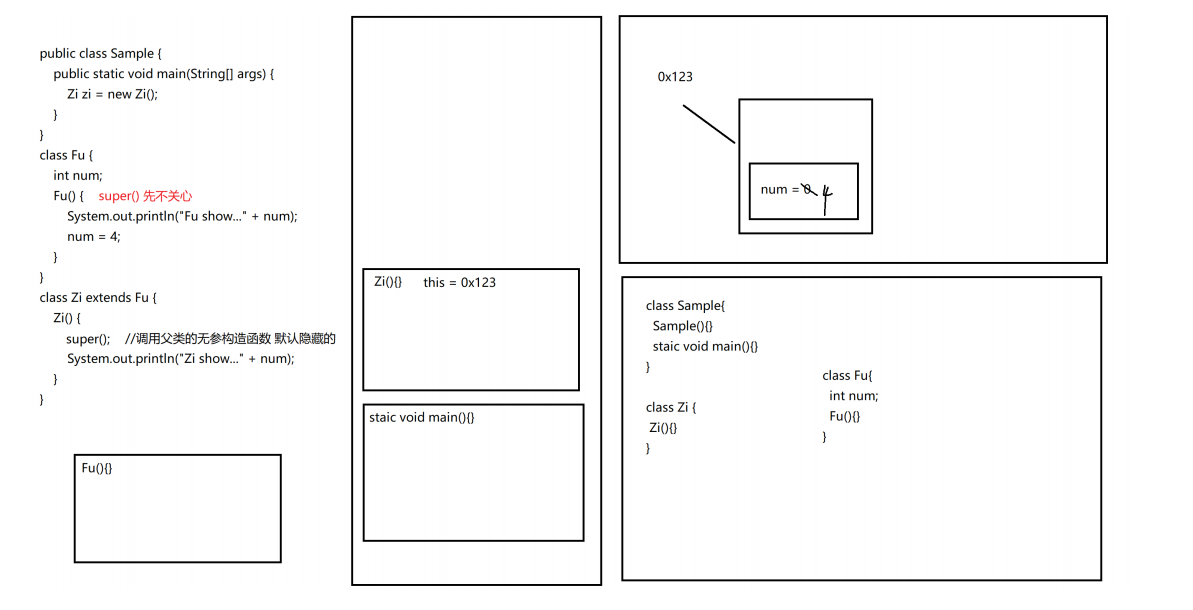### 8.1.4 this()与super()是否冲突

1. 如果子类的多个构造函数们之间没有调用关系，则每一个子类的构造函数第一句都是 super()，即子类的多个构造函数都是先调用父类的构造函数对父类的数据进行初始化。
2. 子类中有 多个构造函数，且存在构造函数之间的调用关系，即构造函数的第一句为this(参数列表),在使用该构造函数创建对象时会调用该类的其他构造函数来调用父类的构造函数。
public class Sample {
public static void main(String[] args) {
Zi zi1 = new Zi();
Zi zi2 = new Zi(1);
Zi zi3 = new Zi(1,2);
}
}
class Fu {
int num;
Fu() {
System.out.println("Fu show...");
}
}
class Zi extends Fu {
Zi() {
this(1);
System.out.println("Zi show ... 0");
}
Zi(int a) {
this(1,2);
System.out.println("Zi show ... 1");
}
Zi(int a , int b) {
super();
System.out.println("Zi show ... 2");
}
}
/*

Fu show...
Zi show ... 2
Zi show ... 1
Zi show ... 0
Fu show...
Zi show ... 2
Zi show ... 1
Fu show...
Zi show ... 2
*/


• 在构造函数中，第一句要么是this()，要么是super()
• 不存在每一个构造函数第一句都是this()，否则递归调用
• 存在每一个构造函数第一句都是super()，构造函数之间不调用
• this()与super()本身不冲突的，如果构造函数之间有调用关系，那么最后一个被调用的构造函数就不能再回调，那么其第一句就不能是this()，只能是super()
• 如果父类中，没有无参构造函数的存在，只有有参数的构造函数的话，那么子类中默认的super() 就调用不到父类无参构造函数！引发错误！
public class Sample {
public static void main(String[] args) {
Zi zi1 = new Zi();
Zi zi2 = new Zi(3);
Zi zi3 = new Zi(5,6);
}
}
class Fu {
int num;
Fu () {
System.out.println("Fu constructor... 0 " + "num = " + num);
num = 10;
}
Fu (int a) {
System.out.println("Fu constructor... 1 " + "num = " + num);
num = a;
}
}
class Zi extends Fu {
int num = 10;
Zi() {
System.out.println("Zi constructor... 0 " + "num = " + num + " fu num = " +super.num);
}
Zi(int a) {
this(a,0);
System.out.println("Zi constructor... 1 " + "num = " + num + " fu num = " +super.num);
}
Zi(int a, int b) {
super(a + b);
num = a + b;
System.out.println("Zi constructor... 2 " + "num = " + num + " fu num = " +super.num);
}
}
/*

Fu constructor... 0 num = 0
Zi constructor... 0 num = 10 fu num = 10
Fu constructor... 1 num = 0
Zi constructor... 2 num = 3 fu num = 3
Zi constructor... 1 num = 3 fu num = 3
Fu constructor... 1 num = 0
Zi constructor... 2 num = 11 fu num = 11
*/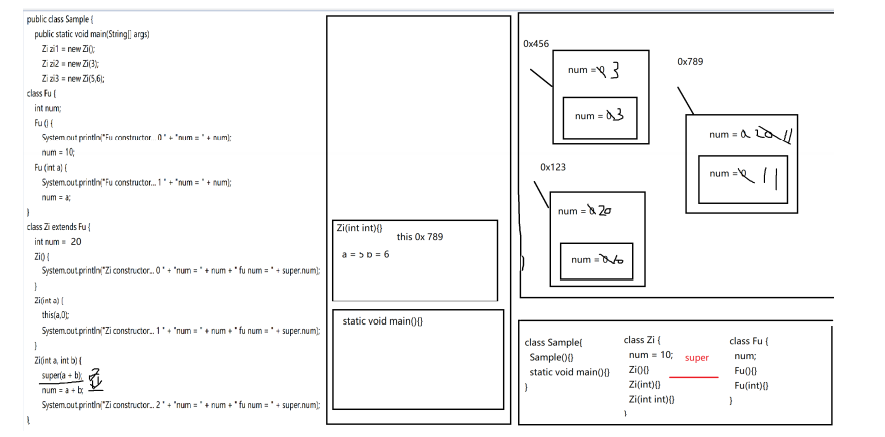### 8.1.5 子父类中，成员函数的特点

public class Sample {
public static void main(String[] args) {
Zi zi = new Zi();
zi.showA();
zi.showB();
zi.showC();
zi.showD();
}
}
class Fu {
void showA() {
System.out.println("Fu showA...");
}
void showC() {
System.out.println("Fu showC...");
}
private void showD() {
System.out.println("Fu showD...");
}
}
class Zi extends Fu{
void showB() {
System.out.println("Zi showB...");
}
@Override
void showC() {
System.out.println("Zi showC...");
}
void showD() {
System.out.println("Zi showD...");
}
}
/*

Fu showA...
Zi showB...
Zi showC...
Zi showD...
*/

• 如果父类有，子类没有，调用的是父类的
• 如果父类没有，子类有，调用的是子类的
• 如果父类有，子类也有，调用的是子类的
• 如果父类有，但是为私有，则子类继承不到，除非子类自己写一个

1. 重写的时候，子类的权限必须大于等于父类的权限，否则编译时报错
2. 重写的时候，返回值类型可以缩小：如父类返回Object，子类重写方法可以返回String

### 8.1.6 重载与重写的区别

1. 同一个类中
2. 方法名相同
3. 参数列表不同
4. 与返回值类型和访问控制权限无关
• 重写
1. 继承关系
2. 方法名相同
3. 参数列表相同
4. 返回值类型可以缩小（如父类返回值为Object类型，子类可以返回String类型）
5. 访问控制权限可以放大
6. 声明异常可以缩小（如父类throws Exception，子类可以声明throws NullPointerException）
public class Sample {
public static void main(String[] args) {
Zi zi = new Zi();
zi.showC(10);
zi.showC(10,20);
}
}
//public > 默认 > protected > private
class Fu {
public void show(){
System.out.println("Fu show...");
}
public int showB() {
return -1;
}
public void showC(int a){
System.out.println("Fu showC...");
}
}
class Zi extends Fu {
void show() {
System.out.println("Zi show...");
}
public void showB() {
}
public void showC(int a,int b){
System.out.println("Zi showC....");
}
}
/*
Sample.java:13: 错误: Zi中的show()无法覆盖Fu中的show()
void show() {
^
正在尝试分配更低的访问权限; 以前为public
1 个错误
*/
/*
Sample.java:21: 错误: Zi中的showB()无法覆盖Fu中的showB()
public void showB() {
^
返回类型void与int不兼容
*/


### 8.1.7 子父类中，静态成员的特点

• 静态变量的特点与成员变量是一致的
• 静态函数的特点与成员函数是一致的
综合案例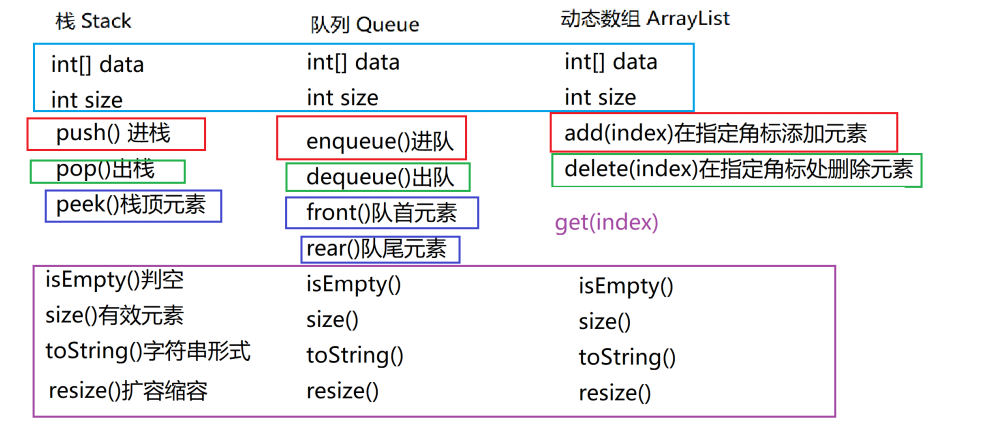public class Sample {
public static void main(String[] args) {
ArrayList list = new ArrayList();
for (int i = 1; i <= 12; i++) {
}
System.out.println(list);
System.out.println(list.get(3));
System.out.println(list.delete(3));
System.out.println(list);

Stack stack = new Stack();
for (int i = 1; i <= 5; i++) {
stack.push(i);
}
System.out.println(stack);
System.out.println(stack.pop());
System.out.println(stack);

Queue queue = new Queue();
for (int i = 1; i <= 5; i++) {
queue.enqueue(i);
}
System.out.println(queue);
System.out.println(queue.dequeue());
System.out.println(queue);
}
}
class ArrayList {
private int[] data;
private int size;
int capacity = 10;

public ArrayList() {
data = new int[capacity];
size = 0;
}

//在指定角标处加入元素e
public void add(int index,int e) {
//1.对index做合法性处理
if (index < 0 || index > size) {
System.out.println(">>>插入位置不合法！");
return;
}
//2.考虑扩容的问题
if (size == data.length) {
resize(data.length * 2);
}
//3.合法加入 别忘了移动元素 不合法 结束并提示
for (int i = size - 1; i >= index; i--) {
data[i + 1] = data[i];
}
data[index] = e;
size++;
}

//删除指定角标处的元素 并返回
public int delete(int index) {
//1.角标的合法性
if (index < 0 || index >= size) {
System.out.println(">>>删除位置不合法！");
return -1;
}
//2.删除即可 别忘了移动元素
int ret = data[index];
for (int i = index + 1; i < size; i++) {
data[i - 1] = data[i];
}
//3.考虑缩容的问题
size--;
if (size == data.length / 4 && data.length > capacity) {
resize(data.length / 2);
}
return ret;
}

//获取指定角标处的元素
public int get(int index) {
//1.考虑角标的合法性
if (index < 0 || index >= size) {
System.out.println(">>>获取位置不合法！");
return -1;
}
//2.直接返回
return data[index];
}

//扩容+缩容的操作
private void resize(int newlength) {
int[] newData = new int[newlength];
for (int i = 0; i < size; i++) {
newData[i] = data[i];
}
data = newData;
}

public boolean isEmpty() {
return size == 0;
}

public int size() {
return size;
}
public String toString() {
//如果size == 0 返回 []
//如果不是[开始 拼接数字 每一个数字后面有个, 但是最后一个数字后面拼接]
String s = "[";
if (isEmpty()) {
s += "]";
} else {
for (int i = 0; i < size; i++) {
if (i == size - 1) {
s += data[i] + "]";
} else {
s += data[i] + ",";
}
}
}
return s;
}
}
class Stack extends ArrayList{
public Stack() {
super();
}
public void push(int e) {
}
public int pop() {
return delete(size() - 1);
}
public int peek() {
return get(size() - 1);
}
}
class Queue extends ArrayList {
public Queue() {
super();
}
public void enqueue(int e) {
}
public int dequeue() {
return delete(0);
}
public int front() {
return get(0);
}
public int rear() {
return get(size() - 1);
}
}
/*

[12,11,10,9,8,7,6,5,4,3,2,1]
9
9
[12,11,10,8,7,6,5,4,3,2,1]
[1,2,3,4,5]
5
[1,2,3,4]
[1,2,3,4,5]
1
[2,3,4,5]
*/


## 8.2 final关键字

final：用于修饰不可改变内容，可以用于修饰类、函数和变量。

• 类：被修饰的类，不能被继承
• 函数：被修饰的方法，不能被重写
• 变量：被修饰的变量，不能被重新赋值

### 8.2.1 final修饰变量

• 基本类型的局部变量，被final修饰后，表示变量所存储的常量值不能改变，只能赋值一次
• 引用数据类型的变量，被final修饰后，只能指向一个对象，地址不能再更改。但是不影响对象内部的成员变量值的修改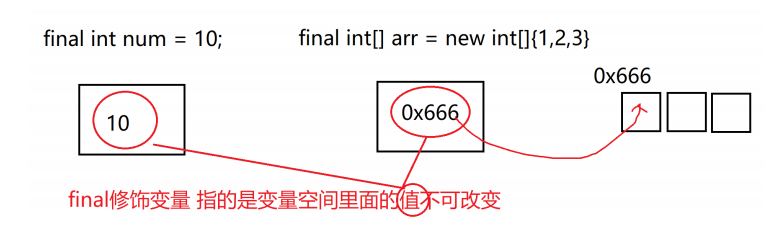public class Sample {
public static void main(String[] args) {
final Demo d = new Demo();
//d = new Demo(); //error
//d.num = 30;//error
d.haha = 40;//ok
}
}
class Demo {
public final int num = 10;
public int haha = 20;
}


public static final 数据类型 变量名 = 常量数据；

public static final int MAX_VALUE = 100;

## 8.3 抽象类

### 8.3.1 概述

• 抽象方法：没有方法体的方法
• 抽象类：包含抽象方法的类

### 8.3.2 抽象方法

修饰符 abstract 返回值类型 方法名 (参数列表)；


### 8.3.3 抽象类

public abstract class Animal {
public abstract void run()；
}


• 抽象类和抽象函数都需要被abstract修饰，抽象方法一定在抽象类中
• 抽象类不能创建对象，因为如果一旦创建对象，在调用其函数时，函数没有具体执行内容
• 只有覆盖了抽象类中所有的抽象函数后，子类才可以实例化。否则，该子类还是一个抽象类

### 8.3.4 抽象类的细节问题

• 抽象类一定是一个父类，因为抽象类本身就是有多个事物进行抽取而来的
• 抽象类有成员变量、成员函数、构造函数，抽象类与一般类唯一的区别就是抽象类中抽象函数，其他一律相同（抽象类不能创建对象）
• 抽象类比一般类可以多定义一个成员：抽象函数
• 一般类可以创建对象，而抽象类不能创建对象
• 有抽象函数的类一定是抽象类，抽象类不一定有抽象函数！
• 抽象关键字abstract不能与以下关键字共存
• final：final修饰类，表示该类不能被继承；final修饰函数时，表示函数不能被重写；不能与absteact共存，抽象类本就是父类，并且其中的抽象函数就等着被子类重写。
• private：private修饰函数，表示函数被私有，不能被子类继承；不能与absteact共存，抽象函数就等着被子类重写。
• static：static修饰的函数，属于类的，随着类的加载从而被加载方法区中，和对象没有关系了，可以直接用类来调用静态成员，如果抽象函数被静态修饰，被类调用时没意义。

## 8.4 接口

### 8.4.1 概述

• 当一个抽象类中，所有的方法都是抽象函数时，那么，该类就可以用接口来表示
• 接口不是一个类了，一些类的功能和操作不再适用于接口
• 接口中没有成员函数，没有成员变量，没有构造函数，没静态函数，没有静态变量
• 接口也不能直接去创建对象

• 接口中的变量 默认是公共静态常量 public static final类型 就算不写这些关键字 也是默认的
• 接口中的函数 默认是公共抽象的函数 public abstract 类型 就算不写这些关键字 也是默认的

### 8.4.1 默认方法的使用

• 一个接口中，有多个默认方法时，实现类都可继承使用。如果默认方法有重名的，必须重写一次。
• 接口中的默认方法可以继承，可以重写，二选一，但是只能通过实现类的对象来调用。
1. 继承默认方法，代码如下：

public interface LiveAble {
public default void fly(){
System.out.println("天上飞");
}
}


public class Animal implements LiveAble {
// 继承，什么都不用写，直接调用
}


public class InterfaceDemo {
public static void main(String[] args) {
// 创建子类对象
Animal a = new Animal();
// 调用默认方法
a.fly();
}
}
/*

*/

1. 重写默认方法，代码如下：

public interface LiveAble {
public default void fly(){
System.out.println("天上飞");
}
}


public class Animal implements LiveAble {
@Override
public void fly() {
System.out.println("自由自在的飞");
}
}


public class InterfaceDemo {
public static void main(String[] args) {
// 创建子类对象
Animal a = new Animal();
// 调用重写方法
a.fly();
}
}
/*

*/


### 8.4.2 静态方法的使用

• 接口中，存在同名的静态方法并不会冲突，原因是只能通过各自接口名访问静态方法
• 静态与.class 文件相关，只能使用接口名调用，不可以通过实现类的类名或者实现类的对象调用，代码如下：

public interface LiveAble {
public static void run(){
System.out.println("跑起来~~~");
}
}


public class Animal implements LiveAble {
// 无法重写静态方法
}


public class InterfaceDemo {
public static void main(String[] args) {
// Animal.run(); // 【错误】无法继承方法,也无法调用
LiveAble.run(); //接口的静态方法使用接口名来调用接口中的静态方法
}
}
/*

*/


### 8.4.3 私有方法的使用

• 私有方法：只有默认方法可以调用
• 私有静态方法：默认方法和静态方法可以调用

public interface LiveAble {
default void func(){
//默认方法可以调用接口中的私有方法和静态的私有方法
func1();
func2();
}
private void func1(){
System.out.println("跑起来~~~");
}
private void func2(){
System.out.println("跑起来~~~");
}
}


### 8.4.4 优先级的问题

interface A {
public default void methodA(){
System.out.println("AAAAAAAAAAAA");
}
}

class D {
public void methodA(){
System.out.println("DDDDDDDDDDDD");
}
}

class C extends D implements A {
// 未重写methodA方法
}

public class Test {
public static void main(String[] args) {
C c = new C();
c.methodA();
}
}
/*

DDDDDDDDDDDD
*/


### 8.4.5 接口与类、接口之间的关系

1. 类与类之间是单继承关系，类与接口之间是多实现关系，接口与接口之间是多继承关系
2. 类与接口之间的实现关系用implements关键字表示，可以实现多个接口，但是类要么实现接口中的所有方法，如果抽象方法有重名的，只需要重写一次，要么这个类声明为abstract
3. 接口与接口有着多继承的关系，对于一个子类接口（继承了其他接口的方法）的实现子类（接口的实现类）而言，要么把这几个接口全部实现，要么声明为abstract，如果父接口中的默认方法有重名的，那么子接口需要重写一次
4. 接口与接口之间不存在实现的关系

• 接口中的变量都是全局静态常量
• 接口中的方法都是全局抽象函数
• 接口含有默认方法和静态方法（JDK 8）
• 接口含有私有方法和私有静态方法（JDK 9）
• 接口中，没有构造方法，不能创建对象
• 接口中，没有静态代码块
• 子类必须覆盖接口中所有的抽象方法，或声明为abstract
• 类与接口之间可以存在多实现关系
• 接口与接口之间可以存在多继承关系
• 类与类之间只能是单继承关系

### 8.4.6 接口与抽象类的区别

• 都位于继承或实现的顶端
• 都不能实例化
• 都包含抽象函数，其子类都必须覆盖这些方法

• 一个类只能继承一个父类，但是可以实现多个接口
• 抽象类中其实可以存在一些已经实现好的方法，有部分未实现的方法由子类来决定；接口中只能包含抽象函数，子类必须完全实现

## 8.5 多态

### 8.5.2 实现多态的前提

1. 继承或者实现【二选一】
2. 方法的重写【意义体现：不重写，无意义】
3. 父类引用指向子类对象【格式体现】

### 8.5.3 多态的体现

父类类型 变量名 = new 子类对象；



Fu f = new Zi();
f.method();


public abstract class Animal {
public abstract void eat();
}

class Cat extends Animal {
public void eat() {
System.out.println("吃鱼");
}
}

class Dog extends Animal {
public void eat() {
System.out.println("吃骨头");
}
}

public class Test {
public static void main(String[] args) {
//多态形式，创建对象，向上转型
Animal cat = new Cat();
//先找父类中是否有eat()这个方法，没有编译报错，有的话调用子类中定义的方法体
cat.eat();

Animal dog = new Dog();
dog.eat();
}
}


### 8.5.4 多态的好处

public abstract class Animal {
public abstract void eat();
}

class Cat extends Animal {
public void eat() {
System.out.println("吃鱼");
}
}
class Dog extends Animal {
public void eat() {
System.out.println("吃骨头");
}
}

public class Test {
public static void main(String[] args) {
// 多态形式，创建对象
Cat c = new Cat();
Dog d = new Dog();
// 调用showCatEat
showCatEat(c);
// 调用showDogEat
showDogEat(d);
/*
以上两个方法, 均可以被showAnimalEat(Animal a)方法所替代
而执行效果一致
*/
showAnimalEat(c);
showAnimalEat(d);
}
public static void showCatEat (Cat c){
c.eat();
}
public static void showDogEat (Dog d){
d.eat();
}
public static void showAnimalEat (Animal a){
a.eat();
}
}


### 8.5.3 引用类型转换

#### 8.5.3.1 向上转型

父类类型 变量名 = new 子类类型();



#### 8.5.3.2 向下转型

子类类型 变量名 = (子类类型) 父类变量名;



abstract class Animal {
abstract void eat();
}

class Cat extends Animal {
public void eat() {
System.out.println("吃鱼");
}
public void catchMouse() {
System.out.println("抓老鼠");
}
}

class Dog extends Animal {
public void eat() {
System.out.println("吃骨头");
}
public void watchHouse() {
System.out.println("看家");
}
}

public class Test {
public static void main(String[] args) {
// 向上转型，只能调用父类中定义的方法，不可以调用子类中定义的方法
Animal a = new Cat();
a.eat(); // 调用的是 Cat 的 eat

// 向下转型
Cat c = (Cat)a;
c.catchMouse(); // 调用的是 Cat 的 catchMouse
}
}


public class Test {
public static void main(String[] args) {
// 向上转型
Animal a = new Cat();
a.eat(); // 调用的是 Cat 的 eat

// 向下转型
Dog d = (Dog)a;
d.watchHouse(); // 调用的是 Dog 的 watchHouse 【运行报错】
}
}


变量名 instanceof 数据类型


public class Test {
public static void main(String[] args) {
// 向上转型
Animal a = new Cat();
a.eat(); // 调用的是 Cat 的 eat

// 向下转型
if (a instanceof Cat){
Cat c = (Cat)a;
c.catchMouse(); // 调用的是 Cat 的 catchMouse
} else if (a instanceof Dog){
Dog d = (Dog)a;
d.watchHouse(); // 调用的是 Dog 的 watchHouse
}
}
}


## 8.6 内部类

### 8.6.2 成员内部类

class 外部类 {
class 内部类{
}
}


class Car { //外部类
class Engine { //内部类
}
}


### 8.6.3 访问特点

• 内部类可以直接访问外部类的成员，包括私有成员
• 外部类要访问内部类的成员，必须要建立内部类的对象

外部类名.内部类名 对象名 = new 外部类型().new 内部类型()；

Outter.InnerB b=new Outter().new InnerB();
Outter.InnerB b=new Outter.InnerB();

public class Person {
private boolean live = true;
class Heart {
public void jump() {
// 直接访问外部类成员
if (live) {
System.out.println("心脏在跳动");
} else {
System.out.println("心脏不跳了");
}
}
}
public boolean isLive() {
return live;
}
public void setLive(boolean live) {
this.live = live;
}
}

public class InnerDemo {
public static void main(String[] args) {
// 创建外部类对象
Person p = new Person();
// 创建内部类对象
Heart heart = p.new Heart();

// 调用内部类方法
heart.jump();
// 调用外部类方法
p.setLive(false);
// 调用内部类方法
heart.jump();
}
}
/*

*/


### 8.6.4 匿名内部类

1. 定义子类
2. 重写接口中的方法
3. 创建子类对象
4. 调用重写后的方法

new 父类名或者接口名(){
// 方法重写
@Override
public void method() {
// 执行语句
}
};


public abstract class FlyAble{
public abstract void fly();
}

public class InnerDemo {
public static void main(String[] args) {
/*
1.等号右边:是匿名内部类，定义并创建该接口的子类对象
2.等号左边:是多态赋值,接口类型引用指向子类对象
*/
FlyAble f = new FlyAble(){
public void fly() {
System.out.println("我飞了~~~");
}
};

//调用 fly方法,执行重写后的方法
f.fly();
}
}


public class InnerDemo2 {
public static void main(String[] args) {
/*
1.等号右边:定义并创建该接口的子类对象
2.等号左边:是多态,接口类型引用指向子类对象
*/
FlyAble f = new FlyAble(){
public void fly() {
System.out.println("我飞了~~~");
}
};

// 将f传递给showFly方法中
showFly(f);
}

public static void showFly(FlyAble f) {
f.fly();
}
}


public class InnerDemo3 {
public static void main(String[] args) {
/*
创建匿名内部类,直接传递给showFly(FlyAble f)
*/
showFly( new FlyAble(){
public void fly() {
System.out.println("我飞了~~~");
}
});
}

public static void showFly(FlyAble f) {
f.fly();
}
}


## 8.7 包与权限

### 8.7.1 包

1. 管理java文件的：方便寻找（包名+类名）、解决重名的问题
2. 保护资源 （结合着访问控制符，来限定资源的访问）

#### 8.7.1.1 包的使用

• 一般都用小写英文
• 见名之义 公司域名的倒写+ 【部门名称】+项目名称+模块 、用.分隔，不能以.开头

package com.openlab.student.entity;

import java.util.Scanner;：类的完全限定名
import java.util.*;：导入util包下的所有java类,但是不会导入子包中的类

### 8.7.2 权限

#### 8.7.2.1 概述

• public：公共的
• protected：受保护的
• default：默认的
• private：私有的
publicprotecteddefaultprivate

public>protected>default>private

• 成员变量使用 private ，隐藏细节
• 构造方法使用 public ，方便创建对象
• 成员方法使用 public ，方便调用方法

## 8.8 枚举类型

### 8.8.1 概述

public enum Role2 {
ROLE_NOMAL,
ROLE_VIP,
ROLE_SUPER_VIP
}


private Role2 role;


Role2 r=user.getRole();
if(r==Role2.ROLE_SUPER_VIP) {
System.out.println("超级用户。。。。。。。。。。。。");
}else if(r==Role2.ROLE_NOMAL) {
System.out.println("普通用户。。。。。。。。。。。。");
}

switch(r) {//byte int short String(1.7+)，enum
case ROLE_SUPER_VIP:
System.out.println("超级用户。。。。。。。。。。。。");
break;
case ROLE_NOMAL:
System.out.println("普通用户。。。。。。。。。。。。");
break;
case ROLE_VIP:
System.out.println("VIP用户。。。。。。。。。。。。");
break;
}


# 九、常用类解析

## 9.1 Object类

### 9.1.1 概述

java.lang.Object类是Java语言中的根类，即所有类的父类。它中描述的所有方法子类都可以使用。在对象实例化的时候，最终找的父类就是Object，如果一个类没有特别指定父类， 那么它默认继承自Object类。

• public boolean equals(Object obj)：指示其他某个对象是否与此对象相等
• public String toString()：返回该对象的字符串表示
• public final native Class<?> getClass()：返回的是对象所属类的字节码对象（类的完全限定名）
• public native int hashCode()：返回对象的哈希值，默认返回对象的地址，允许子类重写
• protected void finalize()：当垃圾回收器确定不存在对该对象的更多引用时，由对象的垃圾回收器调用此方法

### 9.1.2 equals()方法

public boolean equals(Object obj)：指示其他某个对象是否与此对象相等，默认使用==判断两个对象是否相等，其实是比较对象的地址是否相等。

import java.util.Objects;

public class Person {
private String name;
private int age;

@Override
public boolean equals(Object obj) {
// 如果对象地址一样，则认为相同
if (this == obj)
return true;
// 如果参数为空，或者类型信息不一样，则认为不同
if (obj == null || !(obj instanceof Person))
return false;

// 向下转型
Person person = (Person) obj;
// 要求基本类型相等，并且将引用类型交给java.util.Objects类的equals静态方法取用结果
return age == person.age && Objects.equals(name, person.name);
}
}


String类中的equals()方法

String类重写类父类Object类中的equals()方法，它比较的是两个对象的值是否相等。

 public boolean equals(Object anObject) {
//首先判断两个对象的地址是否相等
if (this == anObject) {
return true;
}
//在判断两个传入类是否为String 类，并向下转型后比较值是否相等
if (anObject instanceof String) {
String anotherString = (String)anObject;
int n = value.length;
if (n == anotherString.value.length) {
char v1[] = value;
char v2[] = anotherString.value;
int i = 0;
while (n-- != 0) {
if (v1[i] != v2[i])
return false;
i++;
}
return true;
}
}
return false;
}


### 9.1.3 toString()方法

public String toString()：把当前对象转换成字符串形式，未重写时，返回：类的完全限定名+@+哈希值的十六进制字符串，可以继承，可以重写。

public String toString() {
return getClass().getName() + "@" + Integer.toHexString(hashCode());
}


public class Person {
private String name;
private int age;

@Override
public String toString() {
return "Person{" + "name='" + name + '\'' + ", age=" + age + '}';
}
}


### 9.1.4 getClass()方法

public final native Class<?> getClass()：返回的是对象所属类的字节码对象（类的完全限定名）。

native： 本地的，修饰的方法，没有方法体，但是不代表没有实现，通常是由C/C++来实现的。

Class类 ：字节码文件的类型，也是对象，类只加载一次。

## 9.2 StringBuffer和StringBuilder类

### 9.2.1 字符串拼接问题

public class StringDemo {
public static void main(String[] args) {
String s = "Hello";
s += "World";
System.out.println(s);
}
}


### 9.2.2 概述

StringBufferStringBuilder类都继承于AbstractStringBuilder，内部使用基本的char[] value字符数组来保存字符串，父类为StringBufferStringBuilder提供基本的方法，因此StringBufferStringBuilder类的方法基本相同。

### 9.2.3 StringBuffer和StringBuilder类的不同处

StringBuffer类

• 线程安全的可变字符序列，synchronized 同步锁、线程安全—适合多线程

StringBuilder类

• 不保证同步 ,非线程安全—适合单线程

### 9.2.4 常用的构造方法和方法

• public StringBuilder()：构造一个空的StringBuilder容器。
• public StringBuilder(String str)：构造一个StringBuilder容器，并将字符串添加进去。

• public StringBuilder append(...)：添加任意类型数据的字符串形式，并返回当前对象自身。
• public String toString()：将当前StringBuilder对象转换为String对象。
• public StringBuffer insert(int offset, String str)：指定位置插入字符串。
• public StringBuffer reverse()：翻转该字符串。
• public String substring(int start, int end)：截取指定开始 索引和结束索引位置的字符串。

## 9.3 基本数据类型与包装类

### 9.3.1 概述

Java提供了两个类型系统，基本数据类型引用数据类型，使用基本数据类型在于效率，然而很多情况，会创建对象使用，因为对象可以做更多的功能，如果想要我们的基本类型像对象一样操作，就可以使用基本数据类型对应的包装类，如下：

byteByte
shortShort
intInteger
longLong
floatFloat
doubleDouble
charCharacter
booleanBoolean

### 9.3.2 包装类的继承关系

• Object（顶级父类）
• abstract class Number（父类）
• Byte、Short、Integer、Long、Float、Double（子类）
• Character（子类）
• Boolean（子类）

### 9.3.3 Integer为例

Integer类的定义

• public final class Integer extends Number implements Comparable<Integer>
• final：不能被继承
• extends Number： 继承Number抽象类，实现很多方法，进而具有很多功能
• Comparable<Integer>：实现接口，可以比较
+public int compareTo(T o)：重写比较方法，可以自定义（负数：小于、0：等于、正数：大于）

Integer类中的属性

• MIN_VALUE：-2147483648 =（-2^31）
• MAX_VALUE： 2147483647 =（-2^31 - 1）
• TYPE ：获取int类对象
• SIZE = 32： int数据占32位
• BYTES = SIZE / Byte.SIZE ： int数据占多少字节
• private final int value：存储的就是Integer类的数据，本质上就是int value，value一旦赋值，不能更改
• 直接用常量，可以节约资源

Integer类中的构造方法

• Integer(int value)
• Integer(String s)：要求s必须能转换成int，否则NumberFormatException， 如s=“a123”，报错，源码如下：
 public Integer(String s) throws NumberFormatException {
//parseInt方法在下面
this.value = parseInt(s, 10);
}


Integer类中的常用方法

• static int parseInt(String s)
• static int parseInt(String s, int radix)： 就是把字符串转换成int ,radix进制可选，源码如下:
public static int parseInt(String s, int radix)  throws NumberFormatException {
if (s == null) {
throw new NumberFormatException("null");
}
}
}

int result = 0;
boolean negative = false;
int i = 0, len = s.length();
int limit = -Integer.MAX_VALUE;
int multmin;
int digit;

if (len > 0) {
char firstChar = s.charAt(0);
if (firstChar < '0') { // Possible leading "+" or "-"
if (firstChar == '-') {
negative = true;
limit = Integer.MIN_VALUE;
} else if (firstChar != '+')
throw NumberFormatException.forInputString(s);

if (len == 1) // Cannot have lone "+" or "-"
throw NumberFormatException.forInputString(s);
i++;
}
while (i < len) {
// Accumulating negatively avoids surprises near MAX_VALUE
if (digit < 0) {
throw NumberFormatException.forInputString(s);
}
if (result < multmin) {
throw NumberFormatException.forInputString(s);
}
if (result < limit + digit) {
throw NumberFormatException.forInputString(s);
}
result -= digit;
}
} else {
throw NumberFormatException.forInputString(s);
}
return negative ? result : -result;
}

• boolean equals(Object obj)：当类型相同且值相等，才返回true
public boolean equals(Object obj) {
if (obj instanceof Integer) {
return value == ((Integer)obj).intValue();
}
return false;
}

• static Integer valueOf(int i)
• static Integer valueOf(String s)：把其它数据类型转换成当前类型，源码如下：
public static Integer valueOf(int i) {
//high默认为127，也可以自定义设置值
if (i >= IntegerCache.low && i <= IntegerCache.high)
return IntegerCache.cache[i + (-IntegerCache.low)];
return new Integer(i);
}


1、如果传入的整数是-128-high之间的数，直接从缓存中返回对象（此时地址 相同），否则就新创建一个Integer对象返回（新建一个地址）。
2、Integer类中有一个静态内部类IntegerCache，内部类中有一个cache，当Integer加载的时候，就会初始化此类，缓-128-high（一般就是127）之间的数。
3、 如果你需要Integer类型的对象，优先使用valueOf（先从缓存中读取，节省内存），而不是构造方法。
4、Float、Double类的valueOf（）没有缓存，因为[-128，127] 整数数量是固定的，而小数却是无穷的。

• Byte、Short、Integer、Long的valueOf（）方法都有缓存，【-128，127】，超出范围创建对象
• Float、Double的valueOf（）方法没有缓存，每次都会新创建对象
• Character 的valueOf（）方法有缓存，【0，127】
• Boolean 的valueOf（）方法有缓存，有静态的常量true，false，直接返回

### 9.3.4 Integer类的进制转换

• static String toHexString(int i) ：16进制
• static String toOctalString(int i) ：8进制
• static String toBinaryString(int i)：2进制

### 9.3.5 常用的数据类型转换

• int–>String
• a+""
• String.valueOf()
• Interger.toString(int i)
• String–int
• Integer.parse(String s)
• Integer-String
• Integer.toString()
• String–Integer
• Integer valueOf(String s, int radix)
• Integer(String s)
• int–Integer
• Integer(int i)
• Integer valueOf(int i)
• Integer–int
• int intValue()

### 9.3.6 装箱与拆箱

• 装箱：从基本类型转换为对应的包装类对象。
• 本质：Integer.valueOf()
Integer i=12;//自动装箱
Integer i2=Integer.valueOf(12);
//以上代码效果相同
//-------------------------
Integer num1=12;
Integer num2=12;
System.out.println(num1==num2);//true
/*本质：Integer.valueOf(int i)，先判断该数是否在缓存中，在就返回缓存中的地址，否则就创建新的对象（地址也创建）

*/
//-------------------------
Integer num1=129;
Integer num2=129;
System.out.println(num1==num2);//false
//129没有缓存，每次都需要重写创建，所以num1和num2地址不同
//-------------------------
Double num1=12d;
Double num2=12d;
System.out.println(num1==num2);//false
//因为Double.valueOf(double i) 默认没有缓存

• 拆箱：从包装类对象转换为对应的基本类型。
• 本质：Integer.intValue()
Integer i=12;//自动装箱
int num=i;//自动拆箱

• 不要频繁地装拆箱，浪费内存空间

### 9.3.7 什么时候装箱、拆箱？

Integer num1=400;
int num2=400;
System.out.println(num1==num2);//true
//返回true就可以说明num1发生了拆箱操作。换一句话来说，==链接包装类和基本数据类型的时候，就拆箱

Integer num1=400;
int num2=400;
System.out.println(num1.equals(num2));//true
//返回true就可以说明num2进行了装箱

Long num1=400l;
int num2=400;
System.out.println(num1.equals(num2));//false
//原因：long类型的equals方法要求类型相同且值相同才返回true，而num2自身装箱成了Integer类型

Integer num1=400;
int num2=400;
System.out.println(num1*num2);
//当+、-、*、/、%链接包装类和基本数据类型的时候，是拆箱

//拆箱的时候注意避免空指针异常
Integer num1=null;
int num2=num1;//java.lang.NullPointerException
//拆箱实际在执行intValue()方法


1. 在包装类和基本数据类型中用==+-*/%时会将包装类转换为基本数据类型（拆箱）来进行比较和运算。
2. 在包装类和基本数据类型中调用包装类的方法时，会将基本数据类型转换为包装类（装箱）来判断比较。

### 9.3.8 Character类的常用方法

System.out.println(Character.isDigit('a'));
System.out.println(Character.isLetter('a'));
System.out.println(Character.isWhitespace(' '));
System.out.println(Character.isUpperCase('A'));
System.out.println(Character.isLowerCase('b'));
System.out.println(Character.toLowerCase('U'));


### 9.3.9 BigInteger和BigDecimal

• BigInteger
• 做权限控制
• BigInteger setBit(int n)： bi+2^n
• boolean testBit(int n)：判断n是否在bi+2^n中
public class TestBigInt {

public static void main(String[] args) {
//后台管理员设置
BigInteger bi=new BigInteger("0");
bi=bi.setBit(2);//4 0+2^2
bi=bi.setBit(3);//12  4+2^3
System.out.println(bi);//setBit(n)  bi+2^n

//服务器端判断校验
BigInteger bi2=new BigInteger("36");
System.out.println(bi2.testBit(5));
}
}


• BigDecimal

# 十、异常

## 10.1 异常概述

Exception in thread "main" java.lang.ArithmeticException: / by zero
at Test.main(Test.java:5)

1. 异常名称：java.lang.ArithmeticException
2. 异常出现的原因：/ by zero
3. 异常出现的位置：at Test.main(Test.java:5)

## 10.2 异常体系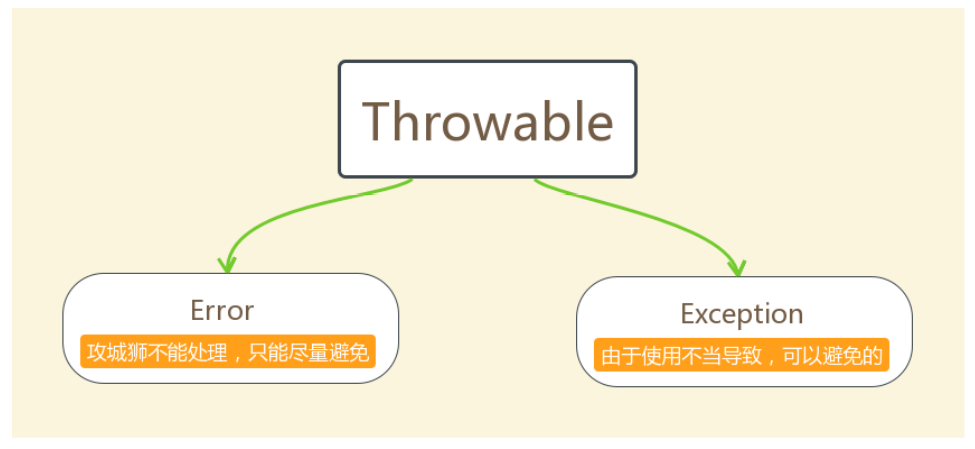Throwable体系

• Error：严重错误Error，无法通过处理的错误，只能事先避免。
• OutOfMemoryError：堆内存溢出（数组定义长度过长）
• StackOverflowError： 栈溢出（递归循环调用）
• Exception：表示异常，异常产生后程序员可以通过代码的方式纠正，使程序继续运行，是必须要处理的。
• RuntimeException：运行时异常，不强制要求程序处理
• ArrayIndexOutOfBoundsException： 数组下标越界异常
• NullPointerException: 空指针异常，对象调用空的属性或方法
• InputMismatchException： 输入不匹配异常
• ArithmeticException： 算术异常
• ClassCastException： 类型转换异常
• NegativeArrayException：数组负下标异常
• StringIndexOutOfBoundsException：字符串索引越界异常
• IOException：输入输出异常
• NumberFormatException：数字格式化异常

Throwable中的常用方法

• public void printStackTrace() ：打印异常的详细信息，包含了异常的类型，异常的原因，还包括异常出现的位置，在开发和调试阶段，都得使用printStackTrace。
• public String getMessage()：获取发生异常的原因，提示给用户的时候，就提示错误原因。
• public String toString()：获取异常的类型和异常描述信息(不用)。

## 10.3 异常分类

• 编译时期异常：checked异常。在编译时期，就会检查，如果没有处理异常，则编译失败，外界的原因，强制要求程序处理。(如日期格式化异常)
• IOException
• ClassNotFoundException
• ParseException
• 运行时期异常：runtime异常。在运行时期，检查异常，在编译时期，运行异常不会被编译器检测(不报错)，不强制要求程序处理。(如数学异常)
• ArrayIndexOutOfBoundsException
• NullPointerException
• InputMismatchException
• ArithmeticException
• ClassCastException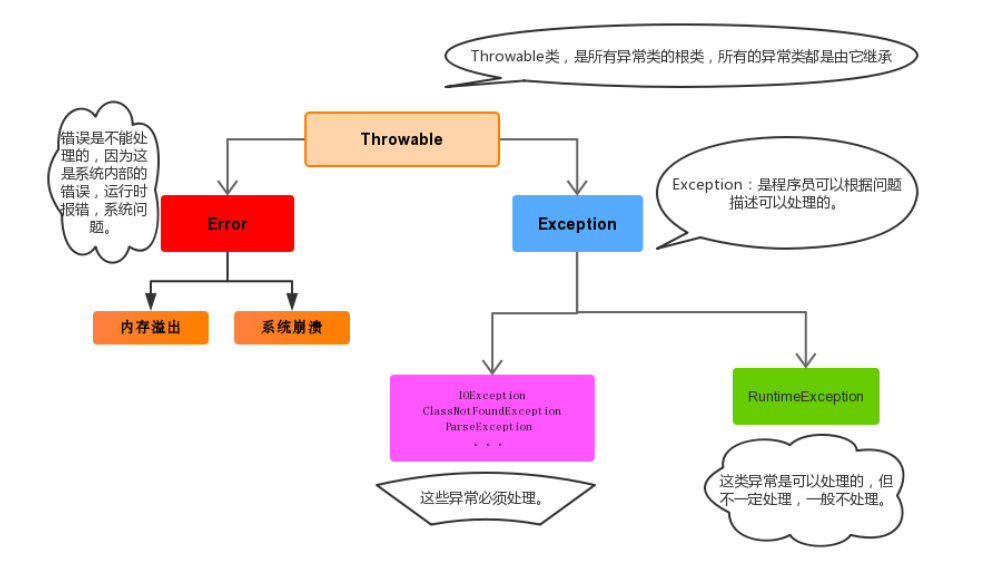## 10.4 异常的处理

1. 方式一：添加判断 if…else
缺点：程序员会把精力放在避免异常，无法集中在业务上了。一直在补漏洞，但是也不一定能补全业务代码，程序被处理异常的代码淹没了，可读性不强。
2. 方式二异常处理机制，预置一些异常处理程序，如果有异常情况，执行相应的处理程序，这样就不会让程序中断。

### 10.4.1 异常处理机制

Java异常处理的五个关键字：trycatchfinallythrowthrows

#### 10.4.1.1 捕获异常try…catch

try{
//可能出现异常的代码
}catch(){
//异常出现的话，如何处理---异常处理程序
//记录日志/打印异常信息/继续抛出异常
}catch(){
//异常出现的话，如何处理---异常处理程序
//记录日志/打印异常信息/继续抛出异常
}
finally{
//不管是否出现异常，都会去执行的代码，关闭资源
}


1. 正常情况下
• 执行try，在执行finally中的代码，然后在执行后续代码
2. 异常情况下
• 出现异常之后的代码不再执行，转到catch块执行，在执行finally中的代码，然后在执行后续代码

catch块的注意点

1. 异常除以可以有多个catch来捕获多个异常，catch是从上往下匹配，上面的匹配上，下面的就不再匹配，所以，catch捕获的异常类型应该从小到大来写，否则会报错，因为有些catch块永远也执行不了。
2. 如果某些异常的处理程序是一样的，可以用|来连接多个异常类型
catch(InputMismatchException|ArithmeticException ex )


#### 10.4.1.2 finally 代码块

finally：有一些特定的代码无论异常是否发生，都需要执行。另外，因为异常会引发程序跳转，导致有些语句执行不到。而finally就是解决这个问题的，在finally代码块中存放的代码都是一定会被执行的。

finally中return的执行流程

• trycatch中都有return语句，先执行return前的语句，再执行finally，最后return
• trycatchfinally中都有return语句，先执行逻辑代码，当执行到finally是直接return。
public class Test4 {
public static void main(String[] args) {
//除法
System.out.println(divide());
}

private static int divide() {
//		int status=0;//0正常  -1 InputMismatchException  -2ArithmeticException
Scanner input=new Scanner(System.in);
try {
System.out.println("请输入一个数");
int num1=input.nextInt();//InputMismatchException  Exception
System.out.println("再输入一个数");
int num2=input.nextInt();//InputMismatchException
int result=num1/num2;//ArithmeticException
System.out.println("结果是"+result);
return 0;
}catch(InputMismatchException ex) {
System.out.println("只能输入数字");
return -1;
}catch(ArithmeticException ex) {
System.out.println("除数不能为0 "+ex.getMessage());
return -2;
}catch (Exception e) {
System.out.println("其它异常");
return -3;
}finally {
System.out.println("程序结束");
}
}
}
/*

12

3

0

a

-1
*/

try {
System.out.println("请输入一个数");
int num1=input.nextInt();//InputMismatchException  Exception
System.out.println("再输入一个数");
int num2=input.nextInt();//InputMismatchException
int result=num1/num2;//ArithmeticException
System.out.println("结果是"+result);
return 0;
}catch(InputMismatchException ex) {
System.out.println("只能输入数字");
return -1;
}catch(ArithmeticException ex) {
System.out.println("除数不能为0 "+ex.getMessage());
return -2;
}catch (Exception e) {
System.out.println("其它异常");
return -3;
}finally {
System.out.println("程序结束");
return -4;
}
/*

3

33

-4
*/


#### 10.4.1.3 抛出异常throw

java中，提供了一个throw关键字，它用来抛出一个指定的异常对象，throw用在方法内，用来抛出一个异常对象，将这个异常对象传递到调用者处，并结束当前方法的执行。

throw new 异常类名(参数);

throw new NullPointerException("要访问的arr数组不存在");
throw new ArrayIndexOutOfBoundsException("该索引在数组中不存在，已超出范围");


#### 10.4.1.3 声明异常throws

修饰符 返回值类型 方法名(参数) throws 异常类名1,异常类名2…{ }


throws用于进行异常类的声明，若该方法可能有多种异常情况产生，那么在throws后面可以写多个异常类，用逗号隔开。

• 运行时异常，不强制要求捕获或者声明。即使声明了，也不要求调用者必须捕获或者声明。所以，我们一般不声明，但是往往会捕获。
• 编译时异常，强制要求捕获或声明。如果方法内部不捕获，那么调用者或者上层调用者就必须捕获。否泽，编译会出错。

## 10.5 异常注意事项

1. 多个异常分别处理。
2. 多个异常一次捕获，多次处理。
3. 多个异常一次捕获一次处理。

try{
编写可能会出现异常的代码
}catch(异常类型A e){ 当try中出现A类型异常,就用该catch来捕获.
处理异常的代码
//记录日志/打印异常信息/继续抛出异常
}catch(异常类型B e){ 当try中出现B类型异常,就用该catch来捕获.
处理异常的代码
//记录日志/打印异常信息/继续抛出异常
}


• 运行时异常被抛出可以不处理。即不捕获也不声明抛出。
• 如果finally有return语句,永远返回finally中的结果,避免该情况.
• 如果父类抛出了多个异常，子类重写父类方法时，抛出和父类相同的异常或者是父类异常的子类或者不抛出异常。
• 父类方法没有抛出异常，子类重写父类该方法时也不可抛出异常。此时子类产生该异常，只能捕获处理，不能声明抛出。

## 10.6 自定义异常

1. 自定义一个编译期异常: 自定义类 并继承于 java.lang.Exception
2. 自定义一个运行时期的异常类:自定义类 并继承于java.lang.RuntimeException

• 可以重写带参构造，设置异常原因。
• 自定义异常只能手动抛出，不能让JVM抛。
// 业务逻辑异常
public class RegisterException extends Exception {
/**
* 空参构造
*/
public RegisterException() {
}
/**
*
* @param message 表示异常提示
*/
public RegisterException(String message) {
super(message);
}
/**
*
* @param cause 表示传入的异常类，可以将异常类进行转换
*/
public RegisterException(Throwable cause) {
super(cause);
}
}


07-05
08-01154
09-29302
07-09105
02-12214
05-081万+
03-3149
05-30
04-12106
02-10324
05-074484
11-141万+
01-03660
05-29107
08-25870

### “相关推荐”对你有帮助么？

•非常没帮助
•没帮助
•一般
•有帮助
•非常有帮助被折叠的  条评论 为什么被折叠?到【灌水乐园】发言veno_冰

¥2 ¥4 ¥6 ¥10 ¥20余额支付 (余额：-- )扫码支付获取中扫码支付点击重新获取扫码支付1.余额是钱包充值的虚拟货币，按照1:1的比例进行支付金额的抵扣。
2.余额无法直接购买下载，可以购买VIP、C币套餐、付费专栏及课程。余额充值